## How to Find the Largest Value in a Column and Return the Adjacent Cell Value in Excel

This post will guide you how to find the largest value in a column and then return the adjacent cell value with a formula in Excel. How do I find the max value and return the cell value from the left adjacent column in Excel.

## 1. Find the Largest Value and Return the Adjacent Cell Value

Assuming that you have a list of data in range A1:B5, and you want to find the largest value from the Sales column and then return the adjacent cell value in product column. How to do it. You can use a formula based on the VLOOKUP function and the MAX function to achieve the result. Like this:

``=VLOOKUP(MAX(A2:A5), A2:B5, 2, FALSE)``

Type this formula into a blank cell and press Enter key on your keyboard.

You should see that the adjacent cell value is extracted.

The A2:B5 is the data range that you want to use in the VLOOKUP function.  And the Number 2 is the column number that the matched value is returned, it should be the product column number.

## 2. Find the Max Value and Return Value from Left Adjacent Cell

If you want to find the max value in Sales column and return value from the left adjacent cell in your worksheet, you need to use the INDEX function to create a formula in combination with the MATCH function and the MAX function. Like this:

``=INDEX(A2:A5,MATCH(MAX(B2:B5),B2:B5,0))``

Type this formula into a blank cell and press Enter key on your keyboard.

## 3. Video: Find the Largest Value in a Column and Return the Adjacent Cell Value in Excel

This video will demonstrate how to use a formula to find the largest value in a column and return the adjacent cell value in Excel.

## 4. Related Functions

• Excel INDEX function
The Excel INDEX function returns a value from a table based on the index (row number and column number)The INDEX function is a build-in function in Microsoft Excel and it is categorized as a Lookup and Reference Function.The syntax of the INDEX function is as below:= INDEX (array, row_num,[column_num])…
• Excel MATCH  function
The Excel MATCH function search a value in an array and returns the position of that item.The MATCH function is a build-in function in Microsoft Excel and it is categorized as a Lookup and Reference Function.The syntax of the MATCH function is as below:= MATCH  (lookup_value, lookup_array, [match_type])….
• Excel MAX function
The Excel MAX function returns the largest numeric value from the numbers that you provided. Or returns the largest value in the array.= MAX(num1,[num2,…numn])…
• Excel VLOOKUP function
The Excel VLOOKUP function lookup a value in the first column of the table and return the value in the same row based on index_num position.The syntax of the VLOOKUP function is as below:= VLOOKUP (lookup_value, table_array, column_index_num,[range_lookup])….

## Convert State Names To Abbreviations

Assume that you got a task to convert the full state’s name into the abbreviations in MS Excel, and for doing this task, you might choose to do it manually, which is an acceptable way only if you don’t have any other way to do it!

But fortunately, there is a way to convert a state’s name into abbreviations, and after reading this article carefully, you would get to know about that way by which you can do this task in a few seconds.

So let’s get straight into it.

## 1. Convert State Names To Abbreviations

#### a. General Formula

The Formula to convert state names to the abbreviations in a matter of seconds is mentioned as follows:

``=VLOOKUP(States_Name,states_Data,2,0)``

#### b. Explanation of Syntax

Before getting knowledge about how to use this Formula to get the work done, we first need to understand how each syntax of the Formula contributes to making this Formula worthful:

• `VLOOKUP`: The VLOOKUP function retrieves data from a range or table row by row.
• `Parenthesis` (): The primary function of this symbol is to group the elements.

#### Let’s See How This Formula Works

A simple formula based on VLOOKUP may be used to convert complete state names to their two-letter abbreviation. The Formula in B2 in the example is:

``=VLOOKUP(A2, states_Data,2,0)``

Where “ states_Data ” refers to the designated E2:F7 range:

This means that this method is based on a database with columns for the full state name and the 2-letter abbreviation. Because we’re using VLOOKUP, we need the whole name in the first column. The table has been dubbed “states_Data” for clarity’s sake.

VLOOKUP is set to retrieve the lookup value from column A. The table array represents the designated range “ states_Data,” The column index is 2 (to extract the abbreviation from the second column). The final option, range lookup, has been set to zero (FALSE).

VLOOKUP finds the matching entry in the “states_Data” database and returns the 2-letter abbreviation.

## 2. Convert Abbreviations to State Names (Lookup In Reverse)

What if you have an abbreviation of the states and want to search up the complete state name in the sample lookup table? You’ll need to use INDEX and MATCH instead in such a situation. With a lookup value in A2, this Formula will yield the whole state name as shown in the lookup table:

``=INDEX(D2:D7,MATCH(A2,E2:E7,0))``

You may use this version to convert a 2-letter abbreviation to a complete state name if you wish to utilize the same-named range “ states_Data.”

``=INDEX(INDEX(states_Data,0,1),MATCH(A1,INDEX(states_Data,0,2),0))``

By specifying a row number of zero, we may utilize INDEX to return whole columns. This is an interesting and helpful aspect of the INDEX function: if you specify zero for the row, you receive complete column.

You may convert abbreviations to complete state names using the INDEX and MATCH methods. The INDEX and MATCH functions can help you out to retrieve the complete state names depending on the abbreviations.

## 3. Video: Convert State Names To Abbreviations

This video will show you how to convert state names to abbreviations in Excel using a formula.

## 4. Related Functions

• Excel VLOOKUP function
The Excel VLOOKUP function lookup a value in the first column of the table and return the value in the same row based on index_num position.The syntax of the VLOOKUP function is as below:= VLOOKUP (lookup_value, table_array, column_index_num,[range_lookup])….
• Excel INDEX function
The Excel INDEX function returns a value from a table based on the index (row number and column number)The INDEX function is a build-in function in Microsoft Excel and it is categorized as a Lookup and Reference Function.The syntax of the INDEX function is as below:= INDEX (array, row_num,[column_num])…
• Excel MATCH  function
The Excel MATCH function search a value in an array and returns the position of that item.The MATCH function is a build-in function in Microsoft Excel and it is categorized as a Lookup and Reference Function.The syntax of the MATCH function is as below:= MATCH  (lookup_value, lookup_array, [match_type])….

## VLOOKUP Returns zero instead of #NA in Excel

This post will guide you how to VLookup and return zero instead of #N/A in Excel. How do I use VLookup function and return zero instead of #N/A if not found in Excel. How to display zero instead of #N/A when using VLOOKUP in Excel. How to replace #N/A with Zero when VLOOKUP.

## 1. VLOOKUP Returns zero instead of #N/A

The VLOOKUP function is one of the most useful function to find data in a given range of cells in Excel. And if the VLOOKUP function cannot find the result that it is looking for, and it will display a #N/A error. And if you would like it to display a “0” instead of #N/A. How to achieve it.

Assuming that you have a list of data in range B1:C7 which contain the product names and sales data, and you want to use Vlookup function to lookup the product “outlook” in range B1:C7, and the return the sales value in sales column.

Type the following formula in a blank cell and then press Enter key in your keyboard.

``=IFERROR(VLOOKUP("outlook",\$B\$1:\$C\$7,2,0),0)``

From the returned result, you can know that the error message #N/A has been replaced with number 0.

Let’s try to look the product “excel ” in range B1:C7, type the following formula in a blank cell:

``=IFERROR(VLOOKUP("excel",\$B\$1:\$C\$7,2,0),0)``

The sales value for product excel has been extracted from the sales column.

## 2. Video:VLOOKUP Returns zero instead of #N/A

This video will show you how to modify the VLOOKUP formula to return zero instead of #NA in Excel.

## 3. Related Functions

• Excel VLOOKUP function
The Excel VLOOKUP function lookup a value in the first column of the table and return the value in the same row based on index_num position.The syntax of the VLOOKUP function is as below:= VLOOKUP (lookup_value, table_array, column_index_num,[range_lookup])….
• Excel IFERROR function
The Excel IFERROR function returns an alternate value you specify if a formula results in an error, or returns the result of the formula.The syntax of the IFERROR function is as below:= IFERROR (value, value_if_error)….

## Excel Vlookup Return True or False

This post will guide you how to vlookup values in a range of cells and then return True or False values in Excel. How to use the VLOOKUP function to vlookup a value in a list of data, and return True or False values in a selected cells.

In Excel, you can use the VLOOKUP function to look for a value in a column in a table and then returns TRUE from a given column in that table if it finds something. If it doesn’t, it returns FALSE.

## 1. Excel Vlookup Return True or False

Assuming that you have a list of data in range of cells A1:A6, and you want to search the range A1:A6 to look for values in range C1:C2. If found, then return True value, otherwise, return False. How to achieve it. You can use the VLOOKUP function in combination with the IF unction and the ISNA function to vlookup value. Like this:

``=IF(ISNA(VLOOKUP(A1,\$C\$1:\$C\$2,1,FALSE)), "FALSE", "TRUE")``

Type this formula in the formula box of Cell B1, and press Enter key in your keyboard. And drag the AutoFill handle over other cells to apply this formula.

## 2. Video: Excel Vlookup Return True or False

This video will introduce you to a simple and effective formula for performing a VLOOKUP function in Excel to search for values in a range of cells and then return True or False values based on the existence of the lookup value.

## 3. Related Functions

• Excel IF function
The Excel IF function perform a logical test to return one value if the condition is TRUE and return another value if the condition is FALSE. The IF function is a build-in function in Microsoft Excel and it is categorized as a Logical Function.The syntax of the IF function is as below:= IF (condition, [true_value], [false_value])….
• Excel VLOOKUP function
The Excel VLOOKUP function lookup a value in the first column of the table and return the value in the same row based on index_num position.The syntax of the VLOOKUP function is as below:= VLOOKUP (lookup_value, table_array, column_index_num,[range_lookup])….
• Excel ISNA function
The Excel ISNA function used to check if a cell contains the #N/A error, if so, returns TRUE; otherwise, the ISNA function returns FALSE.The syntax of the ISNA function is as below:=ISNA(value)….

## Excel Array Construction

This article will talk about how to create one-dimensional array or two-dimensional array by using some functions in Excel. When using array formulas in Excel, we often use functions to construct arrays.

## 1. Generate Array with ROW or COLUMN Functions

Array formulas often need to use “natural number” as parameters of the function, such as the second parameter of the LARGE function, OFFSET function in addition to the first parameter. Through the manual way to enter a constant array will be more trouble, and easy to make mistakes. Then we can use the ROW or COLUMN function in EXCEL to generate a sequence, this method is very convenient and fast.

The following formula produces a vertical array of natural numbers from `1` to `10`.

``{=ROW (1:10)}``

The following formula produces a horizontal array of natural numbers from `1 `to `10`.

``{=COLUMN(A:J)}``

## 2. Generating two-dimensional Array from one-dimensional Array

Below we will show you how to construct a new two-dimensional array with two columns of data.

### a. One-dimensional range rearrangement to generate two-dimensional array

If there is a list of students and the name column of that list contains 10 students’ names, we need to randomly place the students’ names in the name column into the cell range of `5 rows and 2 columns` (a new two-dimensional array).

We can use the following steps to randomize the names of students in `column B` to a `5 row 2 column` range, such as the cell range `E1:F5`.

STEP1# Select the cell range `E1:F5`

STEP2# Enter the following array formula in the formula bar

``=INDEX(B2:B11,RIGHT(SMALL(RANDBETWEEN(A2:A11^0,999)/1%+A2:A11,ROW(1:5)*2-{1,0}),2))``

STEP3# Press CTRL + SHIFT +ENTER shortcut keys to convert the above formula into an array formula.

STEP4# You will see that the students’ names have been randomly placed in a two-dimensional range of array.

Let’s see how this array formula works.

=RANDBETWEEN(A2:A11^0,999)

The RANDBETWEEN function is used to generate an array of 10 values, where the elements are random integers between` 1` and `1000`. Since the elements are randomly generated, the size of the array elements is randomly ordered. The array formula generates an array of random integers as follows.

``={484;203;468;525;702;220;13;163;386;54}``

=RANDBETWEEN(A2:A11^0,999)/1%+A2:A11

The random integer array generated above is multiplied by `100 `and then added to the ordinal array of `1` to `10`. This ensures that the last two digits of the array elements are ordinal numbers `1 `to `10`.

={484;203;468;525;702;220;13;163;386;54}/1%+A2:A11

The above array formula returns the following array:

``{48401;20302;46803;52504;70205;22006;1307;16308;38609;5410}``

=ROW(1:5)*2-{1,0}

The ROW function generates a vertical array` {1;2;3;4;5)`, and then subtracts a constant array {1,0} to produce a two-dimensional array of 5 rows and 2 columns.

=SMALL()

The result is taken as the second argument to the SMALL function, which sorts the array after multiplication and addition processing. Since the original size of the array is random, after sorting, the ordinal number corresponding to the last two digits of each element is randomly ordered.

=RIGHT()

The RIGHT function is used to extract the last two digits of each element, and the INDEX function is used to return the student’s name in the corresponding position in `column B`. In this way, the names in `column B `can be randomly populated into a two-dimensional array of `5 rows and 2 columns`.

### b. Combining two columns of data to create a two-dimensional array

We can use the VLOOKUP function to query from the right to the left, and we can use the array operation and IF function to swap two columns of data to generate a new two-dimensional array.

Here is an example of how the VLOOKUP function can be used to reverse the query by constructing a new array.

If you have a table of employee information, and you need to find the employee’s number by the employee’s name, you can use the VLOOKUP function in combination with the IF function to construct a two-dimensional array to find the corresponding employee number.

The employee information table is as follows:

To find the employee number by name, the steps are as follows.

STEP1# Select the cell `B3`

STEP2# Enter the following formula in the formula bar and press Enter

``=VLOOKUP(B2,IF({1,0},E2:E11,D2:D11),2,)``

STEP3# As you can see, Jerry’s employee number has been found.

Let’s see how the above formula works.

The core part of the formula is `IF ({1,0},E2:E11,D2:D11)`, which uses a horizontal array` {1,0}` and two vertical arrays to perform operations to achieve the position of the column where the employee’s name and work number are swapped. Its returned memory arrays are：

``{"Tom",1;"Jerry",2;"Jacey",3;"Wendy",4;"William",5;"Ocean",6;"scott",7;"charies",8;"ceila",9;"nicole",10}``

The VLOOKUP function then queries the employee’s name in the two-dimensional array generated by the IF function and returns the corresponding employee number.

## 3. Extracting Sub-arrays From Data

In daily work, it is often necessary to extract part of the data from a column and reprocess it. For example, If you want to find out the list of employees who meet the specified requirements in the employee table.

The following describes how to extract some data from a column to form a subarray.

Suppose you have an employee salary table and you want to find out the names of employees whose salary is greater than `\$2000`. The salary table is as follows.

You can refer to the following steps to obtain a list of employees who meet the requirements.

STEP1# First you need to select the cell range` E2:E11`

STEP2# Enter the following formula in the formula bar

``=T(OFFSET(B1,SMALL(IF(C2:C11>2000,A2:A11),ROW(INDIRECT("1:"&COUNTIF(C2:C11,">2000")))),))``

STEP3# Press CTRL + SHIFT +ENTER shortcut keys to convert the above formula into an array formula.

Let’s see how the above formula works.

=IF(C2:C11>2000,A2:A11)

First use the IF function to determine whether the salary meets the conditions, if the salary is greater than \$2000, then return the employee’s ID, otherwise return the logical value FALSE.

=ROW(INDIRECT(“1:”&COUNTIF(C2:C11,”>2000″)))

The COUNTIF function is used to calculate the number of scores greater than `100 `and is combined with the ROW function and INDIRECT function to generate a sequence of natural numbers from `1` to `n`.

=SMALL(IF(C2:C11>2000,A2:A11),ROW( INDIRECT(“1:”&COUNTIF(C2:C11,”>2000″))))

Use the SMALL function to find the employee number whose salary is greater than `\$2000` and return the following memory array.

``={1;3;5;10}``

=OFFSET(B1,{1;3;5;10},)

The OFFSET function extracts the employee’s name from the result returned by the SMALL function and returns the following array of employee names.

``={"Tom";"Jacey";"William";"nicole"}``

=T(OFFSET(B1,{1;3;5;10},))

Finally, the T function is used to convert the multi-dimensional reference returned by the OFFSET function into a memory array.

## 4. Extracting Sub-array from a two-dimensional Array

The cell range `A1:C10` contains data of text and numeric type, see the figure below.

If you want to extract all the text-based data from the specified cell range `A1:C10`, then you can use the following array formula.

``=T(INDIRECT(TEXT(SMALL(IF(A1:C10>="",ROW(A1:C10)/1%+COLUMN( A1:C10)),ROW(INDIRECT("1:"&COUNTIF(A1:C10,"*")))), "r0c00"),))``

Let’s see how the above formula works.

=IF(A1:C10>=””,ROW(A1:C10)/1%+COLUMN( A1:C10))

The IF function is used to determine the type of data in the cell range. If the cell value is the text, then let the cell’s line number multiplied by `100`, and then add the cell’s column number, and then return a numeric result; if the cell value is not text type, then return the logical value `FALSE`.

``={FALSE,102,FALSE;FALSE,202,FALSE;FALSE,302,FALSE;FALSE,402,FALSE;FALSE,502,FALSE;FALSE,602,FALSE;FALSE,702,FALSE;FALSE,802,FALSE;FALSE,902,FALSE;FALSE,1002,FALSE}``

=ROW(INDIRECT(“1:”&COUNTIF(A1:C10,”*”)))

The COUNTIF function is used to calculate the number of text values in the range of cells `A1:C10`, and combined with the ROW function and INDIRECT function to generate a series of natural numbers from 1 to n.

``={1;2;3;4;5;6;7;8;9;10}``

=SMALL(IF(A1:C10>=””,ROW(A1:C10)/1%+COLUMN( A1:C10)),ROW(INDIRECT(“1:”&COUNTIF(A1:C10,”*”))))

The SMALL function is used to extract the position information of the cell where the text is located and return a memory array.

``={102;202;302;402;502;602;702;802;902;1002}``

=INDIRECT(TEXT({102;202;302;402;502;602;702;802;902;1002}, “r0c00”),)

The TEXT function is used to convert the location information to R1C1 reference style, and then use the INDIRECT function to return to the cell reference.

=T(INDIRECT(TEXT({102;202;302;402;502;602;702;802;902;1002}, “r0c00”),))

Finally, the multi-dimensional references returned by the INDIRECT function are converted to memory arrays using the T function.

## 5. Fill the Merged Cells by Array Formula

In the merged cells, only the first cell has a value, while the rest of the cells are empty cells. When we work with the data, we may need to fill the empty cells in the merged cells with the corresponding values to meet the needs of the calculation.

The following is a product sales table, we need to fill the empty cells in the merged cells with the corresponding `region name`. The data table is as follows:

You can fill the data into the merged cells by using the following array formula.

``=LOOKUP(ROW(A2:A13),ROW(A2:A13)/(A2:A13>""),A2:A13)``

Let’s See How This Formula Works:

=ROW(A2:A13)/(A2:A13>””)

This formula assigns a non-empty cell in column A to the row number of that cell, and returns the error value `#DIV/O! `for empty cells, and finally returns a memory array.

``{2;#DIV/0!;#DIV/0!;#DIV/0!;6;#DIV/0!;#DIV/0!;#DIV/0!;10;#DIV/0!;#DIV/0!;#DIV/0!}``

Finally, the LOOKUP function is used to perform a fuzzy search and return the corresponding region name.

## 6. Convert Two-dimensional Array to one-dimensional Array

Some functions only support one-dimensional array as their arguments, not two-dimensional array. For example, the second argument of the MATCH function, the second argument of the LOOKUP function, and so on. If you want to complete the query in a two-dimensional array, you need to first convert the two-dimensional array to a one-dimensional array.

In the figure below, the cell range `A1:C4` is a two-dimensional array, by using the following formula you can return the maximum value less than or equal to `100 `in the cell range; LOOKUP function will perform a fuzzy search from a one-dimensional array returned by the SMALL formula, and return the value that matches the conditions.

The formula is as follows.

``=LOOKUP(100,SMALL(A1:C4,ROW(1:12)))``

Let’s see how this formula works:

=SMALL(A1:C4,ROW(1:12))

Because the cell range is `4 rows and 3 columns`, it is a two-dimensional array containing `12 `elements. You can generate a sequence of natural numbers from `1` to `12 `by using the ROW function. Then use the SMALL function to sort the two-dimensional array and return a one-dimensional memory array. The result is as follows:

``={16;60;78.6;79;97;97;99;#NUM!;#NUM!;#NUM!;#NUM!;#NUM!}``

The LOOKUP function performs a fuzzy lookup by row and ignores the error value `#NUM!`. Finally, the maximum value less than or equal to `100 `is returned, which is `99`.

## How to Look Up the Lowest Value in A List by VLOOKUP/INDEX/MATCH Functions in Excel

VLOOKUP function is very useful in our daily work and we can use it to look up match value in a range, then get proper returned value (the returned value may be just adjacent to the match value). Sometimes we only want to look up the lowest value among all matched values in the list and get its adjacent value, how can we do?

This tutorial will help you to look up the lowest value in a list by VLOOKUP function and User Defined Function with VBA code. However, except VLOOKUP function, we can also use INDEX/MATCH functions together in some situations to look up the lowest value as well. Please see details below.

Precondition:

Prepare a table consists of name, score and range columns. Now we want to get the ‘Range’ of the lowest score.

## 1. Look Up the Lowest Value by VLOOKUP Function

Step1: In E2, enter the formula:

``=VLOOKUP(MIN(B2:B6),B2:C6,2,FALSE)``

As we want to find the ‘Range’ of the lowest score, we need to look up the lowest score among all score first, so we enter MIN(B2:B6) as lookup_value.

Step2: Click Enter to get returned value in C column.

Comment:

a.If there are two duplicate lowest values, VLOOKUP function will look up the first match value and return its adjacent value.

b.This method only works well when returned value is listed in the right column.

## 2. Look Up the Lowest Value using User Defined Function with VBA Code

You can also create a User Defined function with VBA Code to lookup the lowest value in a list to replace the above VLOOKUP formula. Just do the following steps:

Step1: Open your Excel workbook and press Alt + F11 to open the Visual Basic Editor.

Step2: In the Visual Basic Editor, click Insert > Module to insert a new module.

Step3: Copy the below VBA code for the custom function and paste it into the new module. Save the VBA module by clicking File > Save or by pressing Ctrl + S.

```Function MyVLOOKUP_Excelhow(rng As Range, lookup_value As Variant, column_index As Integer, exact_match As Boolean) As Variant
Dim lookup_range As Range
Dim result As Variant
Set lookup_range = rng
lookup_value = Application.WorksheetFunction.Min(lookup_range.Columns(1))
result = Application.WorksheetFunction.VLookup(lookup_value, lookup_range, column_index, exact_match)
MyVLOOKUP_Excelhow = result
End Function
```

Step4: In your Excel worksheet, enter a formula using the custom function just like you would any other built-in Excel function. Enter the following formula in Cell E2:

``=MyVLOOKUP_Excelhow(B2:C6,0,2,FALSE)``

Step5: Press Enter to calculate the result of the formula using the custom function.

## 3. Look Up the Lowest Value by INDEX/MATCH Functions

In above example, if we want to know the name of the lowest score, VLOOKUP function doesn’t work. So we use INDEX and MATCH functions combination to look up the lowest value here.

Step1: In B2, enter the formula:

``=INDEX(A2:A6,MATCH(MIN(B2:B6),B2:B6,0))``

MATCH function returns the location of cell, in this example it returns the lowest value’s row number. Then we can use INDEX function to get proper Name from A2:A6 refer to row number.

Step2: Click Enter to get returned value.

Comment:

a. If there are two duplicate lowest values, INDEX/MATCH functions will look up the first match value and return its adjacent value.

b. This method works well for that returned value lists on the both sides of match value.

## 4. Video: Look Up the Lowest Value in A List

This video will demonstrate how to use the VLOOKUP function and VBA code to look up the lowest value in a list in Excel.

## 5. Related Functions

• Excel INDEX function
The Excel INDEX function returns a value from a table based on the index (row number and column number)The INDEX function is a build-in function in Microsoft Excel and it is categorized as a Lookup and Reference Function.The syntax of the INDEX function is as below:= INDEX (array, row_num,[column_num])…
• Excel MAX function
The Excel MAX function returns the largest numeric value from the numbers that you provided. Or returns the largest value in the array.= MAX(num1,[num2,…numn])…
• Excel MIN function
The Excel MIN function returns the smallest numeric value from the numbers that you provided. Or returns the smallest value in the array.The MIN function is a build-in function in Microsoft Excel and it is categorized as a Statistical Function.The syntax of the MIN function is as below:= MIN(num1,[num2,…numn])….
• Excel VLOOKUP function
The Excel VLOOKUP function lookup a value in the first column of the table and return the value in the same row based on index_num position.The syntax of the VLOOKUP function is as below:= VLOOKUP (lookup_value, table_array, column_index_num,[range_lookup])….
• Excel MATCH  function
The Excel MATCH function search a value in an array and returns the position of that item.The MATCH function is a build-in function in Microsoft Excel and it is categorized as a Lookup and Reference Function.The syntax of the MATCH function is as below:= MATCH  (lookup_value, lookup_array, [match_type])….

## Basic Price Discount Calculation with Excel VLOOKUP Function

We often encounter product discounts in our shopping. Depending on the level of spending, the mall will offer different percentages of discounts. Usually, the more you spend, the bigger the discount, while the less you spend, the smaller the discount.

See the example below. Different discounts are offered depending on the amount of money spent.

With this table, we can calculate our discount based on our actual spending amount. Usually, the value provided in the consumption column is the starting line of the discount. For example, no discount if spending does not reach 1000, and if we spend more than \$3,000 but less than \$5,000, we can get a 15% discount.

In this example, we can use an Excel VLOOKUP function to create a formula to calculate a discount based on a given value. By entering different values, it should be possible to calculate the discount correctly.

## 1. VLOOKUP FORMULA

In Excel, the VLOOKUP function returns a value at a specific location based on the location of the lookup value.

Syntax:

``=VLOOKUP(lookup_value, table_array, column_index_num, [range_lookup])``

So the formula is:

``=VLOOKUP(D2,A2:B6,2)``

### a. VLOOKUP Formula Explanation

In formula =VLOOKUP(D2,A2:B6,2)

#lookup_value: D2

In this example, output discount value is calculated based on the input value, which is stored in cell D2, so the lookup value is D2 (cell reference).

#table_array: A2:B6

All values and discounts are in range A2:B6.

#column_index_num: 2

Discount is in the second column of this table, so column index number is 2.

#range_lookup: empty

Range lookup argument is optional. There are two modes “TRUE” (approximate match) and “FALSE” (exact match). If left blank, it goes into “TRUE” mode, VLOOKUP returns an approximate match. In this example, if we set “FLASE” in formula, we will get #N/A error because there is no “5600” in our table.

Convert cell reference and range reference in the formula bar to the actual value and array. The formula is:

``=VLOOKUP(5600,{1000,0.03;2000,0.05;3000,0.1;5000,0.15;10000,0.2},2)``

As we mentioned above, Excel VLOOKUP runs an approximate match in this case. Since \$5600 is above \$5,000 but below \$10,000, it does not reach the starting line of 20% discount, so it is assigned to the “\$5,000 – 15% discount” level. Thus, a 15 percentage of discount is returned in cell E2, and the 15% is actually retrieved from the Discount column.

• Error #N/A returns by VLOOKUP if input value is lower than \$1000.
• If we change the input value, this formula will calculate the discount accordingly.

### b. VLOOKUP Formula Expand

We can calculate the discount price by simple addition, subtraction, multiplication and division.

## 2. Related Functions

• Excel VLOOKUP function
The Excel VLOOKUP function lookup a value in the first column of the table and return the value in the same row based on index_num position.The syntax of the VLOOKUP function is as below:= VLOOKUP (lookup_value, table_array, column_index_num,[range_lookup])….

## How to Lookup the Next Largest Value

This post will provide you with two different methods to lookup the next largest value in Excel: using a formula and using VBA code. While both methods achieve the same result, they differ in their implementation and can be used depending on your specific requirements.

## 1. Find the Next Largest Value Using Formula

If you want to get the next largest value in another column, you can use a combination of the INDEX function and the MATCH function to create an excel formula.

For example, if we used the VLOOKUP function to lookup the value 200, then it returns “103”, so it returns the value that is less than or equal to the lookup value. but if you want to return the next largest value in the range A2:A5, you can use the following formula:

``=INDEX(A2:A5,MATCH(200,A2:A5)+1)``

And if you want to find the next largest value in another column B2:B5, you just need to change the first argument of the INDEX function, use the range B2:B5 instead of A2:A5, the formula is as follwos:

``=INDEX(B2:B5,MATCH(200,A2:A5)+1)``

Let’s see how the above formula works:

=MATCH(200,A2:A5)+1

The MATCH function return the position of the lookup value 200 in the range A2:A5, if the MATCH function is not find the exact match, then it would return an approximate matched value(it should be less than or equalt to the lookup value) by default, so it returns 1.

To get the position of the next largest value, the position number returned by the match function need to add 1 again. so now we got the position of the next largest value in the range A2:A5. It returns 2.

=INDEX(A2:A5,MATCH(200,A2:A5)+1)

=INDEX(B2:B5,MATCH(200,A2:A5)+1)

The INDEX function returns the value based on the given position that returned by the above MATCH function.

so if you used the Range A2:A5, then the above INDEX function returns the next largest value in the range A2:A5.

And if you used the Range B2:B5, then it returns the next largest value in the range B2:B5.

You can also use the following array formula to achieve the same result.

``=INDEX(B2:B5,MATCH(TRUE,A2:A5>200,0))``

## 2. Find the Next Largest Value with VBA Code (User Defined Function)

You can also create a User defined function with VBA code to find the next largest value in a given range of cells in Excel. Just do the following steps:

Step1: Open your Excel workbook and press “Alt + F11” to open the Visual Basic Editor.

Step2: In the Editor, go to “Insert” > “Module” to create a new module.

Step3: Copy and paste the following code into the new module:

```Function FindNextLargest_ExcelHow(range1 As Range, range2 As Range, lookup_value As Double) As Variant
Dim match_index As Variant
Dim result As Variant
Dim i As Long

On Error Resume Next
match_index = WorksheetFunction.Match(lookup_value, range1, 0)
On Error GoTo 0

If IsError(match_index) Then
FindNextLargest_ExcelHow = CVErr(xlErrNA)
Else
For i = match_index + 1 To range1.Cells.Count
If range1.Cells(i).Value > lookup_value Then
result = range2.Cells(i).Value
Exit For
End If
Next i

If IsEmpty(result) Then
FindNextLargest_ExcelHow = CVErr(xlErrNA)
Else
FindNextLargest_ExcelHow = result
End If
End If
End Function```

Step4: Save the module by going to “File” > “Save” and then close the Editor.

Step5: In your worksheet, select the cell where you want to display the next largest value. Type the following formula into the formula bar:

``=FindNextLargest(A2:A5,B2:B5,200)``

Step6: this formula should return “word”, which is the next largest value in column A after 200.

## 3. Video: Find the Next Largest Value

This video will demonstrate how to lookup the next largest value using both a formula and VBA code.

## 4. Related Formulas

• Find the Relative Position in a Range or Table
If you want to know the relative row position for all rows in an Excel Range (B3:D6), you can use a excel Array formula as follows:=ROW(B3:D6)- ROW(B3) + 1. You can also use another excel array formula to get the same result as follows:=ROW(B3:D6)-ROW(INDEX(B3:D6,1,1))+1…
• Reverse a List or Range
If you want to reverse a list or range, you can use a combination of the INDEX function, the COUNTA function, the ROW function or ROWS function to create a new formula. you can use the following formula:=INDEX(\$A\$2:\$A\$5,COUNTA(\$A\$2:\$A\$5)-ROWS(\$C\$2:C2)+1)…

## 5. Related Functions

• Excel INDEX function
The Excel INDEX function returns a value from a table based on the index (row number and column number)The INDEX function is a build-in function in Microsoft Excel and it is categorized as a Lookup and Reference Function.The syntax of the INDEX function is as below:= INDEX (array, row_num,[column_num])…
• Excel MATCH function
The Excel MATCH function search a value in an array and returns the position of that item.The syntax of the MATCH function is as below:= MATCH  (lookup_value, lookup_array, [match_type])….
• Excel VLOOKUP function
The Excel VLOOKUP function lookup a value in the first column of the table and return the value in the same row based on index_num position.The syntax of the VLOOKUP function is as below:= VLOOKUP (lookup_value, table_array, column_index_num,[range_lookup])….

## Repeat Cell Value N times in Excel

This post will guide you through two different methods to repeat cell values N times in Excel, including using the VLOOKUP function, and VBA code.

## 1. Repeat Cell Value N Times using VLOOKUP Function

Assuming that you have a list of data that contain two column and one column is cell value that you want to repeat, and another column contain N number. To repeat cell value N times in Excel, just do the following steps:

Step1: Insert a column to the left of A, so your current A and B columns are now B and C.

Step2: Put 1 in Cell A1.

Step3: Put =A1+C1 in A2 and drag the AutoFill Handle down to Cell A5.

Step4: Put an empty string in B5, by just entering a single quote (‘) in the cell

Step5: Put a number 1 in E1, a number 2 in E2, and copy down as to get 1, 2, …, 14

Step6: Type the following formula based on the VLOOKUP function into the formula box of cell F1 and then drag the AutoFill Handle over other cells to apply this formula.

``=VLOOKUP(E1,\$A\$1:\$B\$5,2)``

## 2. Repeat Cell Value N Times with VBA Code

You can also use the VBA Code to select a range of cells, then select the destination cell and repeat N time for the selected range of cells based on the next cell in the same row. Just do the following steps:

Step1: Press ALT+F11 to open the Visual Basic Editor.

Step2: Click on Insert > Module.

Step3: Paste the following code into the module.

Step4: Press F5 to execute the code. Or press ALT+F8 to open the Macro dialog box. And select the Macro RepeatCellValuesNTimes_excelhow from the Macro list. Click Run button.

Step5: Select the range of cells that you want to repeat the value in, and click OK.

Step6: Select the destination cell where you want to start repeating the value, and click OK.

Step7: The value will be repeated N times in separate cells.

## 3. Video: Repeat Cell Value N Times in Excel

This video will show you how to repeat cell value N times in Excel using both the VLOOKUP formula and VBA code.

## 4. Conclusion

These methods have its own benefits and drawbacks, so it’s important to choose the one that best fits your needs. Through those two methods, you’ll be able to easily repeat any cell value N times in Excel.

## 5. Related Functions

• Excel VLOOKUP function
The Excel VLOOKUP function lookup a value in the first column of the table and return the value in the same row based on index_num position.The syntax of the VLOOKUP function is as below:= VLOOKUP (lookup_value, table_array, column_index_num,[range_lookup])….

## How to Exclude Values from One Column Based on Values in Another Column in Excel

This post will explain various methods to exclude values from one column based on values in another column in Excel.

Often, while working with large datasets, we need to remove certain values from a column based on criteria from another column. we will cover three different approaches to achieve this: using the VLOOKUP function, the COUNTIF function, and VBA code.

Prepare a table recording the PASS and FAIL status for students. Now we need to exclude students who are listed in PASS column but also listed in FAIL.

## 1. Exclude Values from One Column by VLOOKUP Function

Step1: Insert a new column between column B and column C. Then in C2 enter the formula

``=VLOOKUP(B2,\$D\$2:\$D\$6,1,FALSE)``

Step2: Click Enter. Verify that Ada is displayed in this cell.

Step3: Drag down the fill handle. Verify that C2:C11 are filled with returned values of VLOOKUP function.

Through the screenshot we can see that values need to be removed are displayed in column C.

Step4: Create a filter on C1. Select row 1, click Data in ribbon, select Filter.

Step5: Click arrow button in C1, then Sort & Filter dialog is displayed. Check on Select All option first (this operation will activate all options checked), then uncheck #N/A. Click OK.

Then values need to be excluded are filtered in column B.

Step6: Now you can highlight them or remove them per your request. If you want to highlight them, just select the range marked in red in above screenshot, and click Home->Fill Color button to highlight them.

If you want to remove them, just copy this column to another worksheet, and then filter text by color.

Then delete these filtered cells (press F5 to trigger Go To dialog, and then click Special button, check on Visible cells only option and click OK, all visible cells are selected, then you can delete them). Copy and paste updated column to column B again.

## 2. Exclude Values from One Column by COUNTIF Function

Steps are similar with VLOOKUP function.

Step1: Insert a new column between column B and column C. Then in C2 enter the formula:

``=COUNTIF(\$D\$2:\$D\$6,B2)``

COUNTIF function is used for counting how many times B2 appears in range \$D\$2:\$D\$6. If B2 value appears in the selected range, COUNTIF returns the times B2 appears, if B2 doesn’t appear, COUNTIF returns 0.

Step2: Drag down the fill handle till reaching the last cell in column C. Verify that C2:C11 are filled with returned value 1 or 0.

For values in column B, if 1 is displayed in its adjacent cell of column C, this value should be excluded.

Now you can follow step#4-#6 in method1 to exclude them.

## 3. Exclude Values from One Column Based on Another Column with VBA Code

If you want to exclude values from one column based on values in another column with VBA code, and return an array result, you can use the following steps:

Step1: Open the Excel workbook and press ALT + F11 to open the Visual Basic Editor.

Step2: Click on Insert > Module to add a new module to the workbook.

Step3: Paste the following code into the module. Close the Visual Basic Editor and return to the Excel workbook.

```Sub ExcludeValues_excelhow()
Dim rng1 As Range, rng2 As Range, destRange As Range
Dim arr1 As Variant, arr2 As Variant, result() As Variant
Dim i As Long, j As Long, k As Long, flag As Boolean

' Prompt for the first range of values
Set rng1 = Application.InputBox("Select the first range of values to exclude from:", Type:=8)
If rng1 Is Nothing Then Exit Sub

' Prompt for the second range of values
Set rng2 = Application.InputBox("Select the range of values to exclude based on:", Type:=8)
If rng2 Is Nothing Then Exit Sub

' Prompt for the destination cell
Set destRange = Application.InputBox("Select the destination cell:", Type:=8)
If destRange Is Nothing Then Exit Sub

' Convert the input ranges to arrays
arr1 = rng1.Value
arr2 = rng2.Value

' Exclude values based on the second range
ReDim result(1 To UBound(arr1, 1), 1 To 1)
For i = 1 To UBound(arr1, 1)
flag = False
For j = 1 To UBound(arr2, 1)
If arr1(i, 1) = arr2(j, 1) Then
flag = True
Exit For
End If
Next j
If Not flag Then
k = k + 1
result(k, 1) = arr1(i, 1)
End If
Next i

' Resize the result array
'ReDim Preserve result(1 To k, 1 To 1)

' Write the result to the destination cell
destRange.Resize(k, 1).Value = result
End Sub```

Step4: Press ALT + F8 to open the Macros dialog box. Select the “ExcludeValues_excelhow” macro and click on the “Run” button.

Step5: select the first range of values to exclude from.

Step6: select the range of values to exclude based on.

Step7: select the destination cell to place the result.

Step8: The macro will exclude the values from the first range based on the values in the second range and write the result to the destination cell.

## 4. Conclusion

Now you should have a clear understanding of how to implement each of these methods and choose the one that suits your needs best.

## 5. Related Functions

• Excel VLOOKUP function
The Excel VLOOKUP function lookup a value in the first column of the table and return the value in the same row based on index_num position.The syntax of the VLOOKUP function is as below:= VLOOKUP (lookup_value, table_array, column_index_num,[range_lookup])….
• Excel COUNTIF function
The Excel COUNTIF function will count the number of cells in a range that meet a given criteria. This function can be used to count the different kinds of cells with number, date, text values, blank, non-blanks, or containing specific characters.etc.= COUNTIF (range, criteria)…

## Wedding Budget Template

Setting a wedding budget is one of your wedding planning tasks. You can’t start your wedding process if you’re not sure exactly how much your wedding will cost. We all know that wedding budget planning may be a difficult task, especially for large weddings.

You can refer to the existing wedding budget spreadsheet template to plan your wedding budget, which will make it easy for you to plan your wedding budget. You can refer to the data in the wedding budget template as a guide so that you know exactly how much budget amount you need to allocate in which categories.

You will also need to determine your final wedding budget breakdown based on a number of factors, including where you are getting married and the time of year, as well as your top priorities.

This article will introduce a free Excel wedding budget template that can help you monitor your budget for wedding reception, music, costumes, decorations and more. This wedding budget spreadsheet helps you keep track of your wedding budget by using detailed charts and some tips.

## Wedding budget template

This Wedding budget template is officially provided by Microsoft Excel and you can create the template directly in the Excel application or download it from the official website.

You can use this wedding budget template to track the budget for different affairs in your wedding.

This template contains only one worksheet：Wedding budget. This worksheet will help you keep track of the breakdown of expenses for different items in your wedding.

Let’s see how to use this wedding budget template:

Step1: Open Microsoft Excel Spreadsheet, click on the File menu, and then click on the New submenu

Step2: In the New dialog box, find the template search box, type in the keyword ” Wedding budget template” and press Enter, you will see the Wedding budget template.

Step3: Click the Create button to download the selected Wedding budget template template to create a new Wedding budget template spreadsheet.

Step4: You will need to enter the estimated and actual costs for each expense item in each wedding affairs category table.

Step5: You can see that the estimated total expenses and the actual total expenses for each wedding affairs category are counted automatically in the summary table. The formula is used as follows.

``=VLOOKUP(\$R6,\$M\$6:\$P\$15,3,FALSE)``

If you still need a customized budget template, you can send us a message.

If you are looking for Wedding budget template, check out Microsoft office site by clicking here.

## Build Hyperlink With VLOOKUP in Excel

You might have come across a task in which you were assigned to build hyperlinks, which seems very easy, and if you are new to excel or don’t have enough experience with it, then you might wonder about doing this task manually but let me add that no doubt it seems easy to build two or three hyperlinks manually, but when it comes to building a bulk of hyperlinks, then it becomes a cumbersome task to do it manually moreover you will end up exhausted, and you might not finish the task on time.

But don’t worry about it because we got your back and brought up the easiest way, which is none other than with the aid of the Vlookup Function, which lets you build bulks of hyperlinks within seconds.

So without any delay, let’s dive into it

## General Formula

To create hyperlinks using VLOOKUP, use the formula below.

`=HYPERLINK(VLOOKUP(Lookup_name,Lookup_range,column_number,0), Lookup_name) `

## Explanation Of Syntax

• Hyperlink: The HYPERLINK function creates a clickable hyperlink. This Function can develop connections to workbook locations, internet sites, or files on network servers.
• VLOOKUP: This Function helps lookup data in a range or table row by row. More information on the VLOOKUP function may be found here.
• The comma symbol (,): This symbol is a separator that aids in the separation of a list of values.
• Parenthesis (): This symbol’s Primary Function is to group the elements.

## Summary

You may use the VLOOKUP function in conjunction with the HYPERLINK function to produce a hyperlink from a lookup.

The formula in F5 in the case provided is:

`=HYPERLINK(VLOOKUP(E3,link_range,2,0),E3)`

## Explanation

The hyperlink function allows you to use a formula to build a functioning link. It accepts two parameters: link location and, optionally, familiar name.

VLOOKUP searches for and obtains a link value from column 2 of the designated range “link_range” from the inside out (A3:B5). The lookup value is taken from column E, and VLOOKUP is set to an exact match.

The result is used as link location in HYPERLINK, while column E’s content is used as a friendly name.

As an example,

• To begin, you must prepare sample data in Excel.
• Then, insert the following formula in the formula bar to create a hyperlink from a lookup. Use the Formula
`=HYPERLINK(VLOOKUP(E3,link_range,2,0),E3)`
• To obtain the result, press the Enter key, as illustrated in cell F3.
• Next, insert the following formula in the formula bar to create a hyperlink from a lookup. Use the Formula
`=HYPERLINK(VLOOKUP(E4,link_range,2,0),E4)`
• Finally, press the Enter key to obtain the result, as shown in cell F4 below.

Cell F4, as a result

In another example, we want to retrieve the Link URL from column C based on the Link Name in E3 (Google). We want to build a hyperlink with a name based on those two variables. The outcome is shown in cell F3.

The equation is as follows:

`=HYPERLINK(VLOOKUP(E3, \$B\$3:\$C\$6, 2), E3)`

Cell E3 contains the lookup value. The table array argument has the value range \$B\$3:\$C\$6. Because the range does not change when the formula is duplicated, it must be fixed. Col index num is set to 2, indicating that we want to get data from the second column in the range. Finally, the default value for range lookup is 0 since we want to discover an exact match of “Lookup column” values.

The link location parameter for the HYPERLINK function is the result of the VLOOKUP function. Cell E3 is the friendly name parameter.

To use the HYPERLINK and VLOOKUP capabilities, we must first do the following steps:

Step1: Click on cell F3 to insert the formula:

`=HYPERLINK(VLOOKUP(E3, \$B\$3:\$C\$6, 2), E3)`

Step2:  Enter the formula

Step3:  Drag the formula down to the remaining cells in the column by clicking and dragging the small “+” button at the bottom-right of the cell.

Use of the self-contained VLOOKUP formula

Consequently, the hyperlink for https://www.google.com with the name “Google” will appear in cell F3. The VLOOKUP table shows that this is the Link URL for the term Google from E3.

## Conclusion

This article will teach you to efficiently create hyperlinks using VLOOKUP in MS Excel with examples and pictures.

### Related Functions

The Excel HYPERLINK function creates a shortcut/hyperlink to a document, when you click this hyperlink, the excel will open the file that is stored on a network server or local location.The syntax of the HYPERLINK function is as below:= HYPERLINK(link_location,[friendly_name])…
• Excel VLOOKUP function
The Excel VLOOKUP function lookup a value in the first column of the table and return the value in the same row based on index_num position.The syntax of the VLOOKUP function is as below:= VLOOKUP (lookup_value, table_array, column_index_num,[range_lookup])….

## Calculate Total Cost with Excel VLOOKUP Function

In today’s article we will show you how to calculate the total cost for a given weight using the Excel VLOOKUP function. This function will help us to find the appropriate unit price for that weight and then we can use the simple multiplication “unit price * weight” to get the total cost.

Suppose we want to purchase a batch of something, unit prices are different depending on the weight bands, usually the unit price becomes cheaper as the weight increases.

If the correspondence between weight band and unit price is provided in a table in excel worksheet, how can we find the matching unit price and calculate the total price if we know the weight? Can we just create a simple formula to get the total cost?

Look at the example below. To calculate the total cost of something with a weight of 120kg.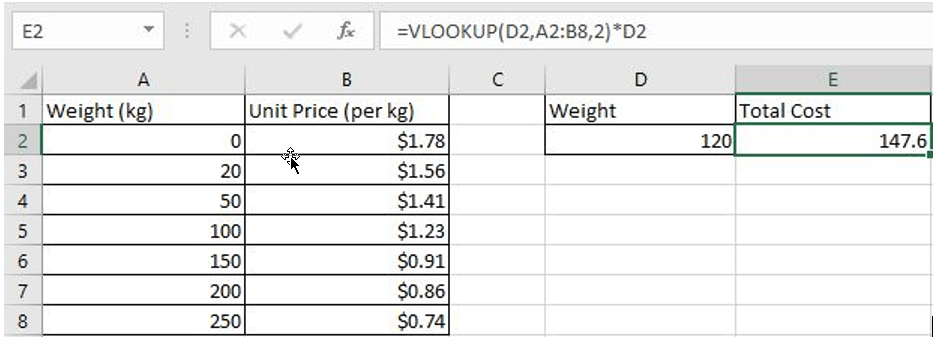## FORMULA

In this example, the formula is:

`=VLOOKUP(D2,A2:B8,2)*D2`

### VLOOKUP INTRODUCTION

VLOOKUP function is a vertical lookup function in Excel, it runs a column-by-column lookup that eventually returns the value corresponding to the looked-up value in the specified column.

Syntax:

`=VLOOKUP(lookup_value,table_array,col_index_num,[range_lookup])`

Arguments:

• lookup_value is the value that needs to be looked up. Make sure it is in the first column of the table. lookup_value can be a numeric value, a reference, or a text string.
• table_array is the table in which the data needs to be looked up. Use a range reference to or a table name to represents the table.
• col_index_num is the column number of the data to be looked up in the table_array.
• range_lookup provides two modes for VLOOKUP; If range_lookup is TRUE or 1, the VLOOKUP function will look for an approximate match; if range_lookup is FALSE or 0, VLOOKUP returns an exact match; and it returns an error value #N/A if not found. If this argument is omitted, the default value is 1, which means that VLOOKUP returns an approximate match.

## EXPLANATION

As we mentioned above, the total cost is equal to the unit price * weight. In this example, the unit price is not a fixed value, it is determined by the weight, and since the weight is given by D2, we need to know the unit price of D2, and we use Excel VLOOKUP function to retrieve the corresponding unit price.

So, in this formula:

VLOOKUP(D2,A2:B8,2) – unit price

D2 – weight of the input

In VLOOKUP(D2,A2:B8,2)

• Lookup_value – D2 (120kg)
• Table_array – A2:B8
• col_index_num – 2 (returns the unit price as unit price in the second column)
• range_lookup – 1- Approximate match (If this argument is omitted, the default value is 1)

### Step#1 VLOOKUP finds the row of entered weight “120”

In this example, “120” is not listed in the first column of the table. Since the value of range_lookup is 1, VLOOKUP returns an approximate match. In approximate match mode, Excel VLOOKUP function will search for “120” in the first column until it encounters the first value greater than it, and then return to the previous row.

In this example, in “Weight” column, Excel VLOOKUP function compares each weight against with the lookup value “120”, and when coming to cell A6, it encounters the first value greater than “120” which is 150, so it returns to A5.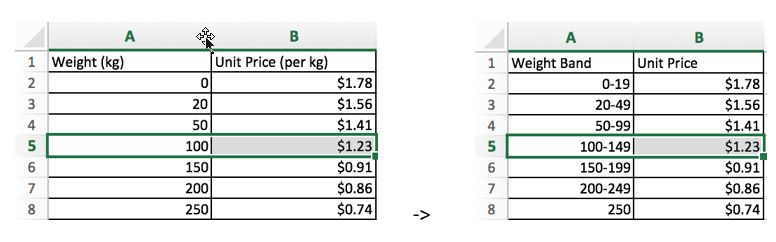(you can compare the two pictures for better understanding the approximate match)

### Step#2 VLOOKUP returns unit price in the row of weight “120”

In the previous step, we know that the row index of weight “120” is 5. Since the column index is 2, Excel VLOOKUP returns the intersection in row 5 and column 2 of table A2:B8. The intersection is B2, value in B2 is 1.23. So VLOOKUP function returns 1.23 finally.

### Step#3 Calculate the formula =VLOOKUP(D2,A2:B8,2)*D2The formula is equivalent to

`=VLOOKUP(120,{0,1.78;20,1.56;50,1.41;100,1.23;150,0.91;200,0.86;250,0.74},2)*120`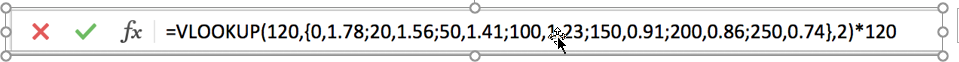In step#2, we know VLOOKUP returns 1.23, so the total cost in this example is 1.23*120=147.6.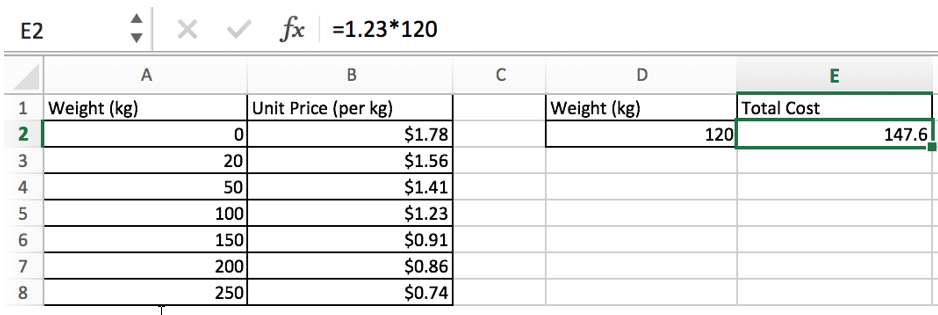### Related Functions

• Excel VLOOKUP function
The Excel VLOOKUP function lookup a value in the first column of the table and return the value in the same row based on index_num position.The syntax of the VLOOKUP function is as below:= VLOOKUP (lookup_value, table_array, column_index_num,[range_lookup])….

## Why Should You Calculate Grades With VLookup Excel?

If you’re looking for a simple way to find out a student’s grades, VLOOKUP Excel can do the trick. This function uses a lookup table to find the values and sort them in ascending order. It also requires the values to be in ascending order. The table itself should be named in the same way as the grades.

VLookup has the ability to adjust grade points for natural breaks in the distribution. This way, you’ll be able to analyze how a student performs on an assignment. Then, you can adjust the grade points and the grade point table. If you’re unsure how to use VLookup Excel, just check out the steps below!

## Calculate Grades With VLOOKUP Excel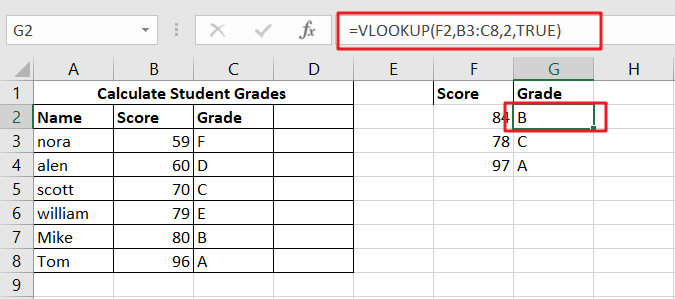If you’re trying to figure out how to calculate grades with VLOOKUP Excel, you’re in the right place. The VLOOKUP function can be used in a variety of ways, from calculating averages to performing a lookup on results. It can also be used to determine a student’s grade, which is particularly useful for educational institutions. However, before you can use VLOOKUP Excel to determine a student’s grade, you need first to figure out the scale of their results.

When you use the VLOOKUP Function in Excel, you’ll need a table with column values sorted in ascending order. Then, you need to set up an “approximate match” condition. The VLOOKUP function works by searching through a table for data. It looks up the value in a column based on its row or column index. The lookup value will return an approximate match when it meets the input value.

### Generic Formula

`=VLOOKUP(score,key,2,TRUE)`

### Syntax And Explanation

`=VLOOKUP(E5,key,2,TRUE)`By putting in the values from the above-given screenshot, we can easily calculate grades using the Vlookup function!

### Summary

VLOOKUP is an Excel function that will look up values from another table and return the value in the same cell. In this example, the result is the grade of student X. The formula can be pasted in any cell, even next to other students. If the formula has too many variables, you can use the Fill Down function from the Edit menu. The VLOOKUP function is easy to use and will save you a lot of time.

## Conclusion

If you’re wondering whether you should use VLOOKUP for calculating grades, you may want to read this article. It will show you the advantages of using the function to make this type of calculation. It will also help you find grades for all students using the same criteria, such as name or exam level. This type of formula will update automatically with any changes to the table. To use VLOOKUP, you’ll need to set up a table with scores in it. You can then sort the data by column and enter a formula that will assign each grade.

### Related Functions

• Excel VLOOKUP function
The Excel VLOOKUP function lookup a value in the first column of the table and return the value in the same row based on index_num position.The syntax of the VLOOKUP function is as below:= VLOOKUP (lookup_value, table_array, column_index_num,[range_lookup])….

## Find and Replace Multiple Values

This post will guide you how to find and replace multiple values at once with VBA macro or using formula  in Excel. How do I make multiple find and replace in Excel.

Suppose that you have a few cells containing few values and you want to find and replace two or three values; then you might think that it’s not a big deal; because you would prefer to do it with the help of the Find and Replace built-in feature of excel, then congratulation because you have supposed to choose the right way for this task.

There isn’t any doubt that you can find and replace values in excel by its` Find and Replace` built-in feature, which works entirely perfect for two or three values, but when you would have the bulk of values, and you want to find and replace them, then doing this task manually by the aid of this `Find and Replace `built-in feature would be a stupid decision because you would get tired of it and would never complete your work on time.

But there isn’t any need to worry about it because, luckily, there are a few effective methods for finding and replacing multiple values in a few seconds.

After reading this article carefully, you would get to know the several ways to find and replace multiple words, individual characters, or strings so that you can select the best one according to your needs and ease.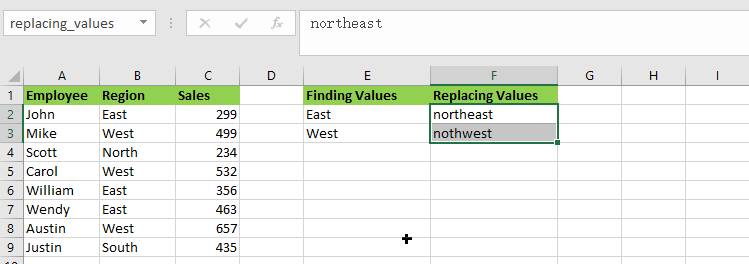So let’s get straight into it!

## Find and Replay Multiple Values using Formula

### General formula:

The formula given below would help you out for finding and replacing multiple values within few seconds:

`=SUBSTITUTE(SUBSTITUTE(B2,INDEX(finding_values,1),INDEX(replacing_values,1)),INDEX(finding_values,2),INDEX(replacing_values,2))`

or

`=SUBSTITUTE(SUBSTITUTE(B2,finding_values,replacing_values), finding_values, replacing_values))`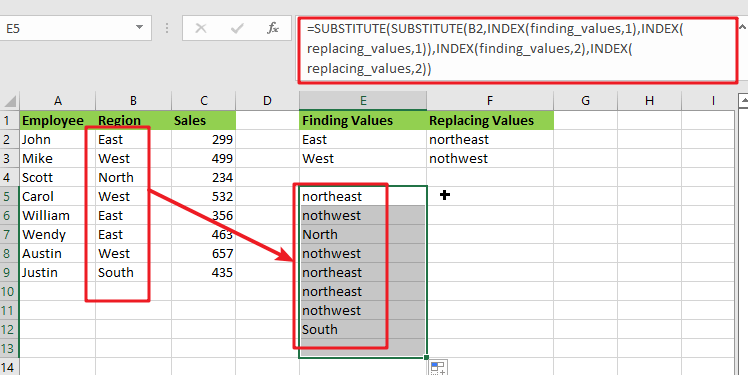### Syntax Explanation:

Before we dive into the formula for getting the job done effectively, we need to understand each syntax so that we can know how each syntax helps to find and replace the multiple values:

• `SUBSTITUTE`: This function lets users replace current text in a text string with new text when they desire to replace text based on its content rather than its position.
• `INDEX`: The INDEX function in Excel is used to repeat characters a specified number of times.
• `B``2`: This is the input value.
• `Find`: This command specifies the text to be found in the input range.
• `Replace`: It represents the text that is being replaced.
• `The comma sign` (,): is a separator that may separate a list of values.
• `Parenthesis` (): The primary purpose of the parenthesis () symbol is to organize the components.

### Let’s See How This Formula Works:

As I said above, there are a few ways to find and replace multiple values in Excel in a matter of seconds, and one of the most popular and simple methods is to utilize the nested SUBSTITUTE function.

The logic of this formula is very simple: you write a few individual functions to replace an old value with a new one. Then you nest those functions one inside the other so that each subsequent SUBSTITUTE uses the output of the previous SUBSTITUTE to find the next value.

`=SUBSTITUTE(SUBSTITUTE(Text_values,finding_values,replacing_values), finding_values, replacing_values))`

Assume you wish to replace the region names in the `B2:B9` list of places with the replacing names.

For getting the desired output, input the old values in` E2:E3` and the new values in `F2:F3`, as seen in the image and formula below. Then, in `E5`, enter the following formula:

`= SUBSTITUTE(SUBSTITUTE(B2, E2, F2), E3,F3)`

You’ll have completed all of the replacements at once:

Please remember that the above method only works in Excel 365, which supports dynamic arrays.

In pre-dynamic versions of Excel 2016, Excel 2019, and prior,type the following formula:

`= SUBSTITUTE(SUBSTITUTE(B2, \$E\$2, \$F\$2), \$E\$3,\$F\$3)`

Also, note that in this case, we lock the replacement values with absolute cell references to ensure that they do not shift when copying the formula down.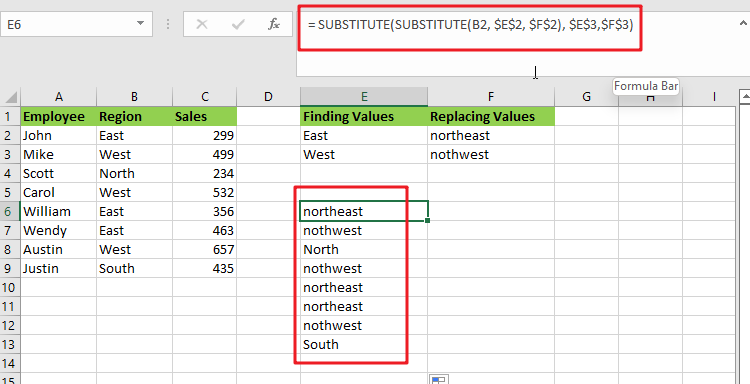The SUBSTITUTE function is case-sensitive, so you must type the old values (old text) in the same letter case as the original data.

This method has a significant drawback: It should only be used for a limited number of find/replace values. This nested function becomes extremely difficult to manage when you have dozens of values to replace.

Benefits: Simple to deploy; compatible with all Excel versions

### Find and replace multiple values using XLOOKUP Function

The XLOOKUP function is very efficient and helpful while replacing the entire cell content rather than just a portion of it.

Assume you have a list of region in column B and want to replace all the “East” or “West” regions as “northeast” or “northwest” values. Enter the following formula in B2:

`=XLOOKUP(B2,\$E\$2:\$E\$3,\$F\$2:\$F\$3,B2)`

The formula does the following when translated from Excel to human language:

It searches the B2 value (lookup value) in E2:E3 (lookup array) and returns a match from F2:F3 (return array). If the original value cannot be retrieved, take it from B2.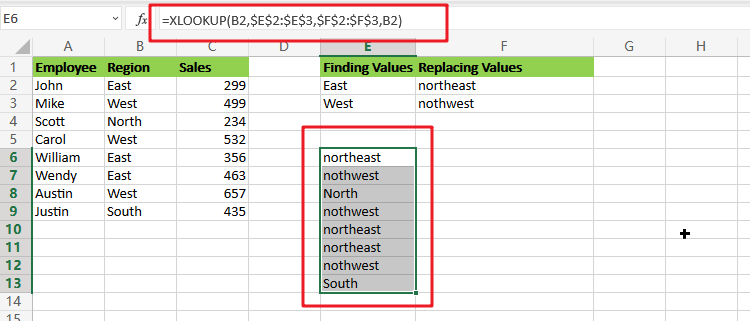Because the XLOOKUP function is only accessible in Excel 365. However, you can easily replicate this behavior using a combination of IFERROR or IFNA and VLOOKUP:

`=IFNA(VLOOKUP(B2,\$E\$2:\$F\$3,2,FALSE),B2)`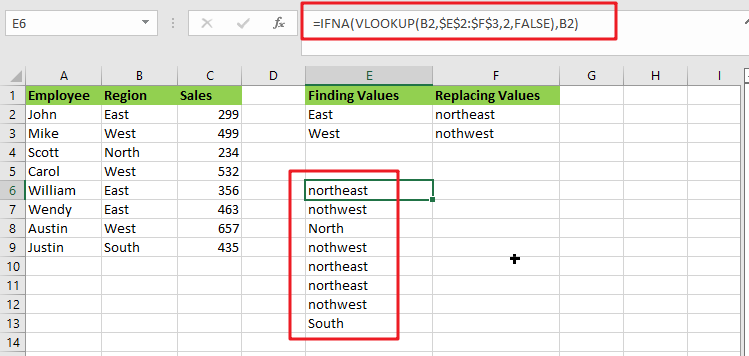Unlike the SUBSTITUTE, the XLOOKUP and VLOOKUP functions are both not case-sensitive, which means they search for lookup values regardless of letter case.

## Find And Replace Multiple Values with VBA Macro

Assuming that you have a list of data in range B1:B6, and you want to find multiple values and replace those value with different values. For example, find “excel” string and replace its as excel2013, and find “word’’ string and replace its as word2013, and so on. How to achieve it. You should use an excel VBA macro to quickly find and replace multiple values. Just do the following steps:

#1 open your excel workbook and then click on “Visual Basic” command under DEVELOPER Tab, or just press “ALT+F11” shortcut.#2 then the “Visual Basic Editor” window will appear.

#3 click “Insert” ->”Module” to create a new module.#4 paste the below VBA code into the code window. Then clicking “Save” button.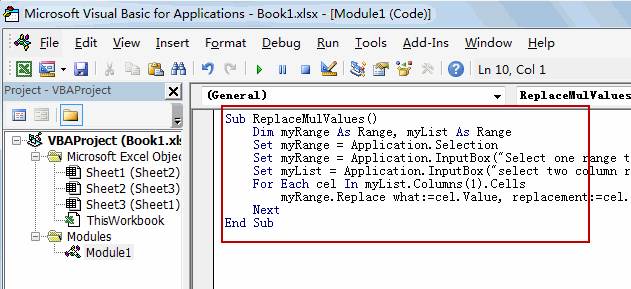```Sub ReplaceMulValues()
Dim myRange As Range, myList As Range
Set myRange = Application.Selection
Set myRange = Application.InputBox("Select one range to be searched", "Find And Replace Multiple Values", myRange.Address, Type:=8)
Set myList = Application.InputBox("select two column range where find/replace pairs are:", "Find And Replace Multiple Values", Type:=8)
For Each cel In myList.Columns(1).Cells
myRange.Replace what:=cel.Value, replacement:=cel.Offset(0, 1).Value
Next
End Sub```

#5 back to the current worksheet, then run the above excel macro. Click Run button.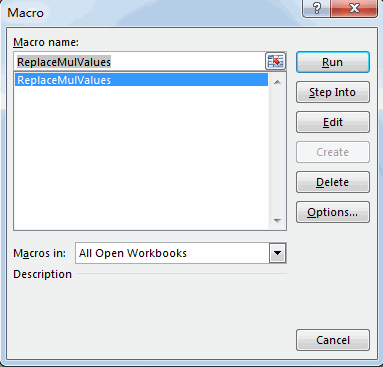#6 Select one range to be searched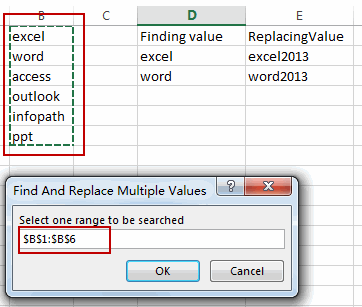#7 select two column range where find/replace pairs are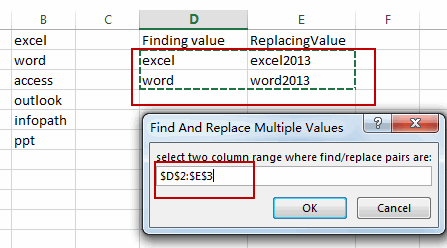#8 let’s see the result.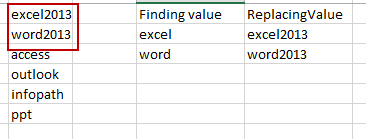### Related Functions

• Excel Substitute function
The Excel SUBSTITUTE function replaces a new text string for an old text string in a text string. The syntax of the SUBSTITUTE function is as below:= SUBSTITUTE  (text, old_text, new_text,[instance_num])….
• Excel IFERROR function
The Excel IFERROR function returns an alternate value you specify if a formula results in an error, or returns the result of the formula.The syntax of the IFERROR function is as below:= IFERROR (value, value_if_error)….
• Excel VLOOKUP function
The Excel VLOOKUP function lookup a value in the first column of the table and return the value in the same row based on index_num position.The syntax of the VLOOKUP function is as below:= VLOOKUP (lookup_value, table_array, column_index_num,[range_lookup])….
• Excel INDEX function
The Excel INDEX function returns a value from a table based on the index (row number and column number)The INDEX function is a build-in function in Microsoft Excel and it is categorized as a Lookup and Reference Function.The syntax of the INDEX function is as below:= INDEX (array, row_num,[column_num])…
• Excel XLOOKUP function
This tutorial will show you how to filter out or extract the top n values from the list having few values using Filter function or XLookup function in Excel The syntax of the xlookup function is as below:=XLOOKUP(lookup value, lookup array, return array, [if not found], [match mode], [search mode])…

## Excel XLOOKUP Function

Excel XLOOKUP Function was added into Excel as a beta feature in August 2019 and is now accessible exclusively in Microsoft 365. (as of July 2021). However, if you fall into this category and often deal with big sets of data in Excel, understanding this method is worthwhile. In this lesson, we will demonstrate how to use the XLOOKUP function to significantly simplify your data search.

## What exactly is Excel XLOOKUP formula?

The Excel XLOOKUP function is a member of the family of lookup and reference functions. It is one of the most helpful features of Microsoft’s famous spreadsheet program. XLOOKUP is the simplest method for finding particular data items inside a cell range. The items included inside a previously set cell range are formatted. Does this sound familiar? The VLOOKUP function likewise operates on this concept. However, the more versatile Excel-XLOOKUP function allows you to look up not just one, but several items. Additionally, you may search for values vertically and horizontally inside your sheet.

In practice, what does this mean? Consider the following scenario: you have a digital client database in the form of an Excel file and are seeking for the address and phone number of a certain individual. With XLOOKUP, you can now search for related items by name and instantly see the needed information. It makes no difference if the sought data are included in a column, row, or table on another page. This indicates that the XLOOKUP function supersedes not only the VLOOKUP but also the HLOOKUP functions.

## XLOOKUP syntax Function

The syntax of the XLOOKUP function is identical to those of VLOOKUP and HLOOKUP. If you’ve ever used them, you’ll appreciate how much more convenient XLOOKUP is. The following is the Excel syntax for the XLOOKUP function:

`=XLOOKUP(lookup value, lookup array, return array, [if not found], [match mode], [search mode])`

The XLOOKUP function accepts up to six parameters, the values of which are shown below.

• `lookup value` (required): the value you’re looking for.
• `lookup array` (required): the array in which the lookup value should be located.
• `return array` (required): the array from which you want to retrieve and return the values when the lookup value is discovered.
• `[if not found]` (optional): If no match is discovered, this value is returned.
• `[match mode]` (optional): This option specifies the sort of match that you are searching for. There are several ways to specify it:
• `0` – It searches for an exact match, and the result must precisely match the lookup array value. When not specified, it is also set as the default.
• `-1` – It searches for an exact match and then returns to the next lower value.
• `1` – It searches for an exact match and then advances to the next bigger number.
• `2` – It performs partial matches using wildcards, where the characters *,?, and have particular significance.

[search mode] (optional): Used to specify the lookup array’s XLOOKUP search mode. There are several ways to specify the same thing:

• 1– Begin the search with the first item. When nothing is supplied, it is defaulted to default.
• -1 – Conducts a reverse search, beginning with the last item.
• 2 – Conducts a binary search in the lookup array in which the data must be sorted ascending. If the data is not properly organized, it may result in mistakes or incorrect outcomes.
• 2 – Conducts a binary search in the lookup array, sorting the data in ascending order. If the data is not properly organized, it may result in mistakes or incorrect outcomes.

XLOOKUP’s Advantages and Disadvantages In Excel, the XLOOKUP function retains some of its benefits over VLOOKUP and INDEX/MATCH. However, it does have certain drawbacks.

1. It operates in both vertical and horizontal directions.
2. Three inputs are required, rather than the four required by the VLOOKUP and INDEX MATCH methods.
3. By default, an exact match is used.
4. Utilize wildcards to conduct partial match lookups.
5. Can execute descending order lookups.

INDEX MATCH now uses a single function rather than two.

## The XLOOKUP Function’s Drawbacks

1. Optional arguments may seem complicated to novices.
2. Can take longer when two ranges are selected and the spreadsheet has an excessive number of cells.
3. When the lookup and array results are not the same length, an error is returned.
4. Both lookup and return ranges must be remembered.

## How to Use Excel’s XLOOKUP Function

The XLOOKUP function is comparable to Excel’s LOOKUP function. XLOOKUP may be used by simply specifying the cell references for the function to act on.

Alternatively, you may utilize the top-level “Formula bar” box to input the XLOOKUP function syntax.

## What makes XLOOKUP superior?

XLOOKUP simplifies and reduces the likelihood of errors in Excel’s most frequently used formulas. Simply type `=XLOOKUP(what you're looking for, the list, the result list)` and you’ll receive the result (or a `#N/A` error if the value is not found).

By default, this function looks for an exact match: One of the drawbacks of VLOOKUP is that you must provide FALSE as the last argument in order to get the right result. XLOOKUP corrects this by defaulting to precise matches. If desired, you may alter the lookup behavior using the match mode option.

The fourth parameter is used to accommodate the value not found situation. To suppress errors in the majority of business cases, we are compelled to encapsulate our lookup formulae with IFERROR or IFNA formulas. XLOOKUP has a fourth argument (described in further detail below) that allows you to choose the default output to use if your value is not found.

XLOOKUP includes extra parameters for searching for unusual circumstances. You may search from the top or bottom of a list, use wildcards, or use quicker alternatives for searching sorted lists.

It outputs a reference, not the value. While this may seem insignificant to casual Excel users, professional Excel users’ eyes light up when they find a formula that returns references. That is, you may mix XLOOKUP results with other formulae in novel ways.

It’s so much cooler to type; all you have to do is type` =XL`. I’m not sure whether this is a lucky coincidence, but saying `=XL` generates this formula.

### Related Functions

• Excel IFERROR function
The Excel IFERROR function returns an alternate value you specify if a formula results in an error, or returns the result of the formula.The syntax of the IFERROR function is as below:= IFERROR (value, value_if_error)….
• Excel VLOOKUP function
The Excel VLOOKUP function lookup a value in the first column of the table and return the value in the same row based on index_num position.The syntax of the VLOOKUP function is as below:= VLOOKUP (lookup_value, table_array, column_index_num,[range_lookup])….
• Excel INDEX function
The Excel INDEX function returns a value from a table based on the index (row number and column number)The INDEX function is a build-in function in Microsoft Excel and it is categorized as a Lookup and Reference Function.The syntax of the INDEX function is as below:= INDEX (array, row_num,[column_num])…
• Excel MATCH  function
The Excel MATCH function search a value in an array and returns the position of that item.The MATCH function is a build-in function in Microsoft Excel and it is categorized as a Lookup and Reference Function.The syntax of the MATCH function is as below:= MATCH  (lookup_value, lookup_array, [match_type])….

## VLOOKUP Formula | Faster Trick with 2 VLOOKUPS

This post will guide you how to use 2 VLOOKUPS function to looking up data entries from a given range of cells in Microsoft Excel. VLOOKUP with 2 lookups can be faster than a single VLOOKUP in certain scenarios. The lookup times will, of course, depend on the data and the indexes.

The generic formula is as follow:

=IF(VLOOKUP(lookup_value,table_array,1,TRUE)=id, VLOOKUP(lookup_value,table_array,column_index,TRUE), NA())

Notes:

• This formula works very well if you have more than a few records. However, when speed really counts, use it with large data set.
• For this excel trick to work, you must sort the data by looking up a specific value.
• The following example uses absolute references. If you don’t want to use this, use named ranges instead!

## Exact-match VLOOKUP Work Slow

Using the VLOOKUP function in “exact match mode” can be time-consuming when working with large data sets. For example, if you have 50 thousand or 100k records, it could take minutes just to do one lookup!

The exact match is determined by supplying FALSE or zero as the fourth argument:

`=VLOOKUP(lookup_value,table_array,column_index,FALSE)`

Using VLOOKUP in this mode will take forever because the program must check every single record until a match is found. This process of searching through all those data points can sometimes be referred to as linear search or even worst-case scenario: quadratic runtime!

## Approximate-match VLOOKUP Works Efficiently and Quickly

Using the VLOOKUP function in “approximate match mode” is much more efficient when dealing with large data sets because it can quickly work through most of your records and return close-to-correct results.

Sometimes, this type of search is called a binary search since you search for approximate matches, not exact ones. The binary search is determined by supplying TRUE or 1 as the fourth argument:

`=VLOOKUP(lookup_value,table_array,column_index,TRUE)`

This simple change can literally speed up your worksheet calculations time by a factor of 100, where it would have taken minutes or hours to get a result with VLOOKUP.

The VLOOKUP function in “approximate match mode” can be faster than a single VLOOKUP because the program only needs to read and process half of your data at each iteration.

## Problem with VLOOKUP Formula

VLOOKUP is a great tool for finding information in tables, but it can produce unexpected results when used without caution. For example, without using an exact match, VLOOKUP can return incorrect results when:

1. Data is sorted differently than the order of references in your formula, or data with similar-looking content is not sorted in the same way.
2. Your table has empty cells, and you did not anticipate this. Please see my previous article on VLOOKUP errors for more information on this topic.

These problems can be problematic when you need to get the exact information every time, especially when working with data sets that are either large or growing larger.

One way to avoid this is by doing two lookups to find an approximate match first and then verifying the result with an exact-match VLOOKUP.

## Creating Formula to Perform 2 Lookups

This trick will work best when the key value sorts the data you are looking up, so using the same order in your formulas makes sense. The generic form of this formula is:

`=IF(VLOOKUP(lookup_value,table_array,1,TRUE)=id, VLOOKUP(lookup_value,table_array,column_index,TRUE), NA())`

This formula uses two lookups and will return “a match” when there is no. Another common alternative to using the last argument of False (exact match) for the first lookup is the Lookup_value argument instead.

## Clarification

VLOOKUP is an incredible tool for finding exactly what you need. For instance, if I were looking up the value of `STU ID` in this example:

`=VLOOKUP(\$F\$2,\$A\$1:\$C\$12,1,TRUE)= \$F\$2`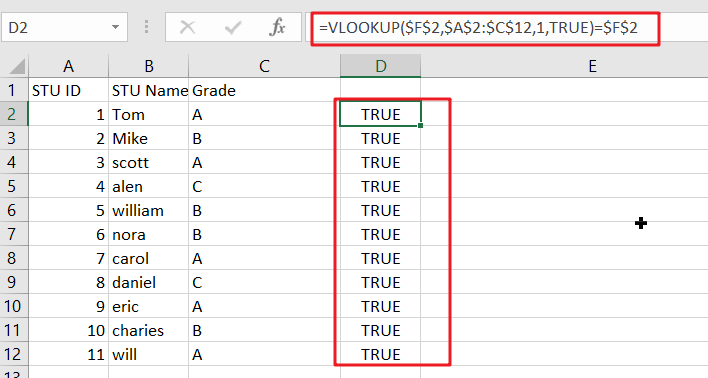This looks up a value in an indexed array and returns TRUE only when the lookup is found. In that case, it uses VLOOKUP again with approximate match mode turned on to get you what’s left of your data:

`VLOOKUP(\$F\$2,\$A\$1:\$C\$12,2,TRUE)`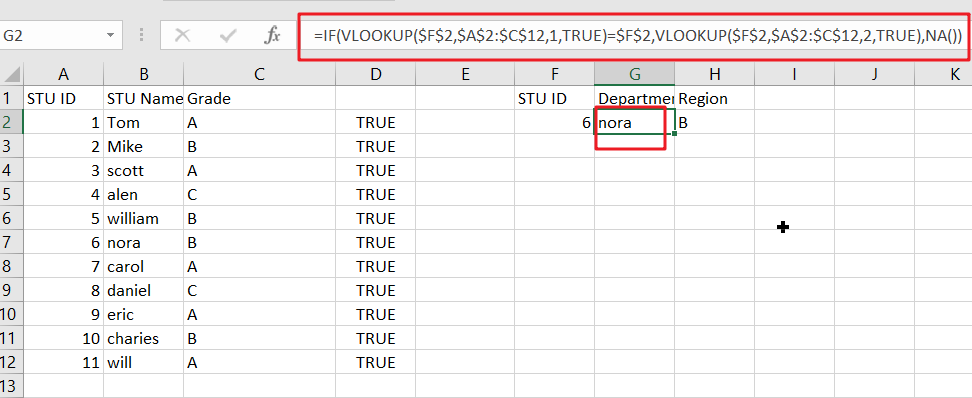Or

`VLOOKUP(\$F\$2,\$A\$1:\$C\$12,3,TRUE)`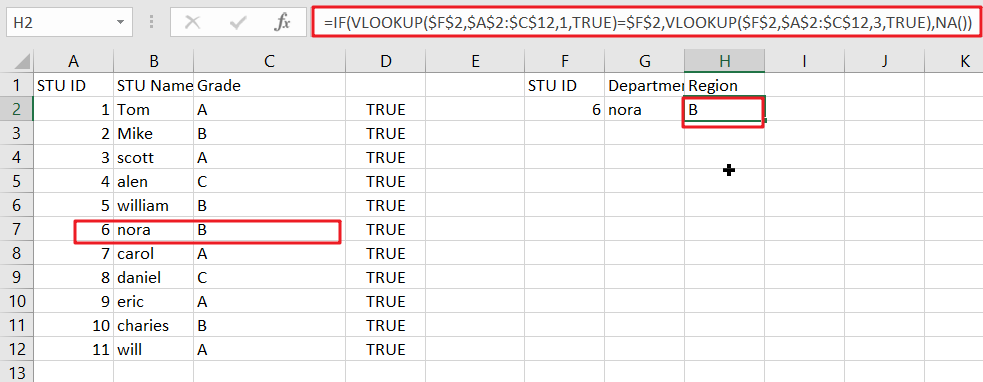No need to worry about missing values since we have already checked the first part of this formula.

If the value isn’t found, then an error will be returned. For this example, we use NA(), which returns #N/A, but you could also return messages like ” Missing” or “Not Found”. This means that whatever value we put in place of “value” will be returned when it’s not found, such as “Missing” or Not Found.”

### Related Functions

• Excel IF function
The Excel IF function perform a logical test to return one value if the condition is TRUE and return another value if the condition is FALSE. The IF function is a build-in function in Microsoft Excel and it is categorized as a Logical Function.The syntax of the IF function is as below:= IF (condition, [true_value], [false_value])….
• Excel VLOOKUP function
The Excel VLOOKUP function lookup a value in the first column of the table and return the value in the same row based on index_num position.The syntax of the VLOOKUP function is as below:= VLOOKUP (lookup_value, table_array, column_index_num,[range_lookup])….
• Excel NA function
The Excel NA function returns the #N/A error value. And the #N/A is the error value that means “no values is available”. The syntax of the NA function is as below:= NA ( )….

## VBA Macro For VLOOKUP From Another Sheet

In the previous post, you should know that how to fix or remove the #N/A error when using VLOOKUP formula to lookup value from another sheet. And this post will show you how to use VBA code to vlookup data from another worksheet in Excel 2013/2016/2019.

## VLOOKUP Function in VBA Macro

You should know that VLOOKUP is a worksheet function in Excel, and it can be used to search a value in an array table and return its corresponding value from another column or from another worksheet in Excel. and the VLOOKUP function can be used in VBA Macro in Excel. The functionality of VLOOKUP function is similar to the functionality in VBA. Both method and the arguments remain the same for VLOOKUP method in VBA code.

## VLOOKUP Example in Excel VBA Example

The below will show you one examples of the VLOOKUP function in Excel VBA Macro. And suppose you want to retrieve the number of sales for product “word” in Cell E2 appears in the second column of the table array argument A2:C6. To lookup for the value “word”, and you just need to follow the below steps:

Step 1: On current visible worksheet, right click on sheet name tab to load Sheet management menu. Select View CodeMicrosoft Visual Basic for Applications window pops up.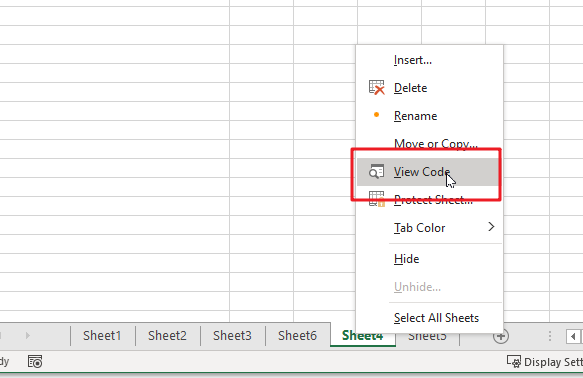Or you can enter Microsoft Visual Basic for Applications window via Developer->Visual Basic. You can also press Alt + F11 keys simultaneously to open it.Step 2: In Microsoft Visual Basic for Applications window, enter below code:

```Sub vlookupExample()

On Error Resume Next

lookupValue = "word"

Set myrange = Range("B2:C6")

result = Application.WorksheetFunction.VLookup(lookupValue, myrange, 2, False)

MsgBox "the sales number of " & lookupValue & " is " & result

End Sub```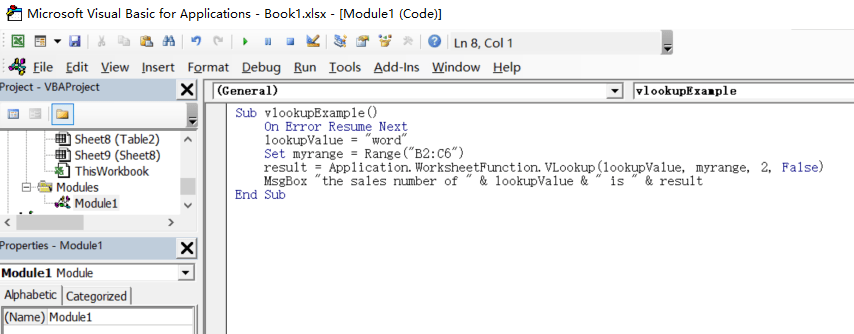Step 3: Save code, quit Microsoft Visual Basic for Applications.

Step 4: Click Developer->Macros to run Macro.Step 5: Select the Macro Name ‘vlookupExample’ from the Macro window and click Run.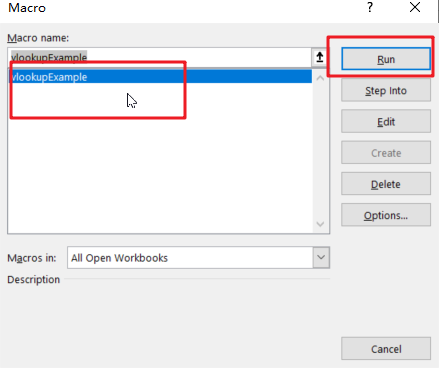Step6: let’s see the result: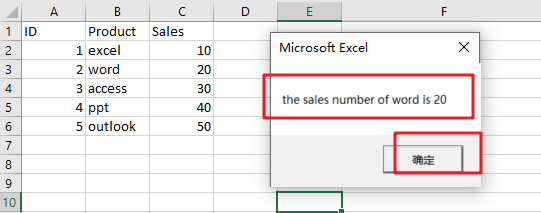Let’s see how this VBA Macro works:

You need to define a lookup value variable, which is the value to lookup.

lookupValue = “word”

Then you need to define the range in which the value and the return value exist. As your array table is B2:C6 in your worksheet, and defining a variable named as “myrange” as below:

Set myrange =Range(“B2:C6”)

Next, you need to call the VLOOKUP function from Application class and its sub-method WorksheetFunction, like below:

Application.WorksheetFunction.VLookup(lookupValue, myrange, 2, False)

Last, you can call MsgBox function to print the result in a message box, so you can use the following line:

MsgBox “the sales number of ” & lookupValue & ” is ” & result

### Related Functions

• Excel VLOOKUP function
The Excel VLOOKUP function lookup a value in the first column of the table and return the value in the same row based on index_num position.The syntax of the VLOOKUP function is as below:= VLOOKUP (lookup_value, table_array, column_index_num,[range_lookup])….

## VLOOKUP From Another Sheet Not Working

In the previous post, you should know that how to fix or remove the #N/A error when using VLOOKUP formula to lookup value from another sheet. And this post will show you reasons why your VLOOKUP formula is not working in Excel 2003/2010/2013/2016 or Excel 365. You should be want to know some of the common reasons why you are getting those VLOOKUP errors, such as:  #N/A, #VALUE, #REF, and returning incorrect results.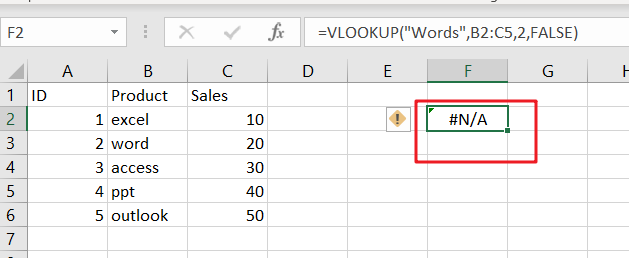## Most of Common VLOOKUP Formula Errors

You should know that VLOOKUP function is a very popular and powerful used function in Microsoft Excel or Google Sheets. And most of users are also complain that the VLOOKUP function fails to work sometimes, and want to know how to fix any VLOOKUP not working problem while using VLOOKUP function to lookup value from another sheet in Excel. Let’s see some common VLOOKUP Formula errors below:

### 1. VLOOKUP #N/A Error

When using VLOOKUP formula to lookup one value in your table array, and if it cannot find an exact match or approximate match, then the #N/A error message would be displayed.  #N/A error means “The Value is not available” in Excel. this error is the most common VLOOKUP error message while looking up values from another sheet or different sheet and returns an incorrect value.

It is not only one reason that the lookup value is not available, and there should be some reasons whey VLOOKUP formula is not working and returns errors in Excel.

#1 Lookup Value Not in the First Column

For VLOOKUP function, the Lookup value must be in the first column or the leftmost column of the table_array argument. If lookup value is not available in the first column of a table array, and the VLOOKUP formula will generate an #N/A error.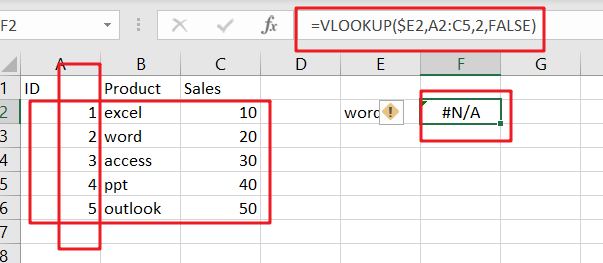If you want to fix this VLOOKUP error in your worksheet, and you need to re-arrange your column range correctly in your table array parameter in VLOOKUP formula. for the above VLOOKUP formula example, you need to change your table_array range from A2:C5 as B2:C5 in VLOOKUP function.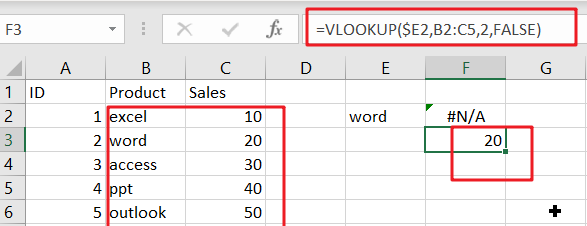#2 Using an Approximate Match

The range_lookup is an optional argument in the VLOOKUP function. And the value can be set as True or False. The default value is True. If you don’t specify this argument and Excel will assume that you want to use an approximate match. And if you want to use an Exact Match in VLOOKUP function, and you should set the fourth argument as FALSE.

Assume that the range_lookup argument is set to “FALSE” or using an approximate match in your VLOOKUP formula, if you want to lookup value from another sheet or same sheet using VLOOKUP function, and lookup value is smaller than the smallest value available in the first column of table array, and your VLOOKUP function should be generated an #N/A error.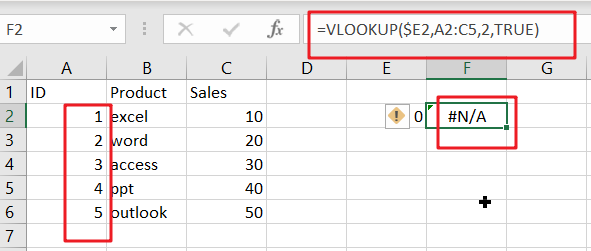#3 Numbers Stored or Formatted as Text

When your data is imported from an external data table or your numeric values were stored as text format, and you try to lookup a numeric value using VLOOKUP function, Excel will generate an error message #N/A.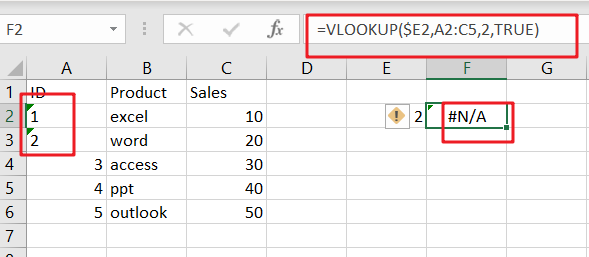If you want to fix this error message for VLOOKUP formula, and you have to check and format all numeric values as Number format. you can select those values, and go to “HOME” tab, and select “Number” format from the drop-down list in the Number category.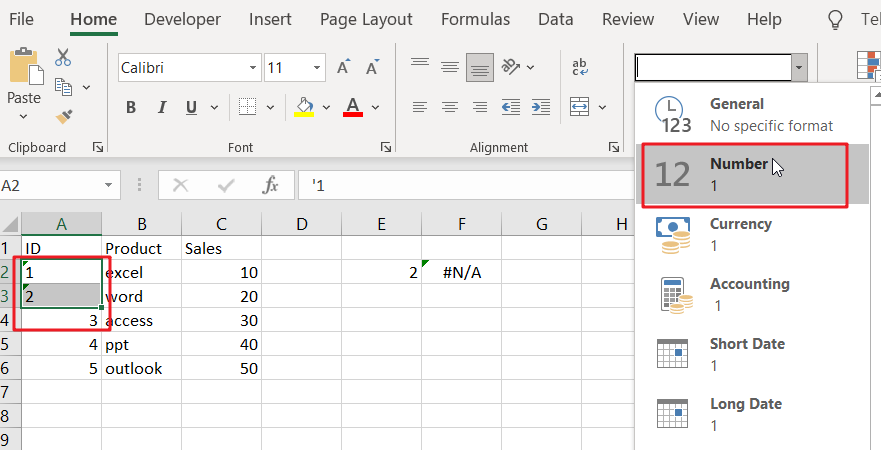#4 Type Mistake for Lookup Value

The VLOOKUP function will use the lookup value as keyword to search for in the lookup table in your current worksheet or in another worksheet, if you mistyped the lookup value and the VLOOKUP function will not find what you typed and Excel will return you an error message #N/A.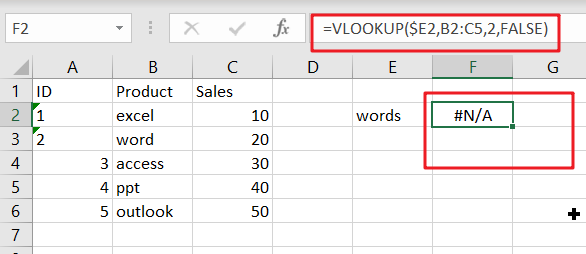For example, if you want to type the lookup value “word”, but mistyped as “words”, and the VLOOKUP function would display the #N/A error message. When the keyword was typed correctly, and the formula should be able to find the expected value.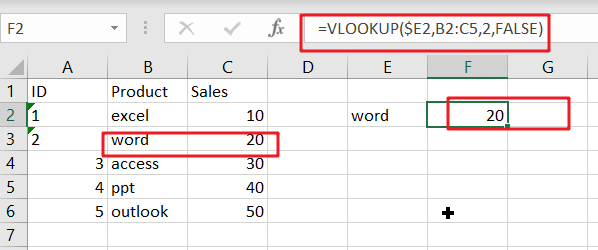#5 Extra Space or Characters (Leading and trailing spaces for Lookup value)

If the lookup value contains extra space or characters in the formula, and the VLOOKUP function will also generate an error message #N/A. the #N/A error is caused by extra spaces which your eyes can hardly see, and everything looks good.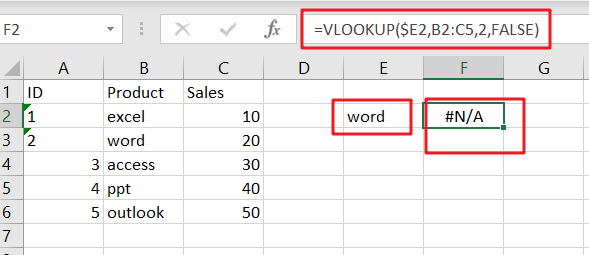To fix or remove this error, and you should check for extra spaces in the formula or remove leading and trailing spaces in the lookup value using TRIM function.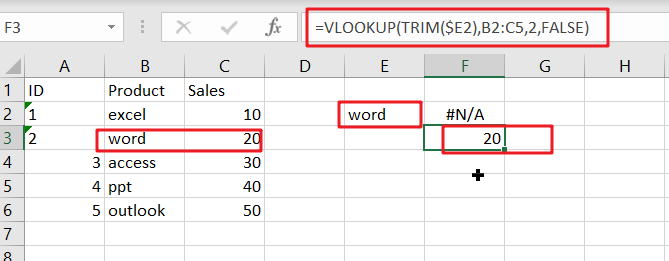### 2. VLOOKUP #REF error

#1 Count the Wrong column number for Column_index_num parameter

If you count the wrong column number for Column index number parameter in the third argument of the VLOOKUP function, and it will also generate an error message #N/A. To fix this error, you need to recount the COLUMNS count from the table array that you are getting your data in your VLOOKUP formula.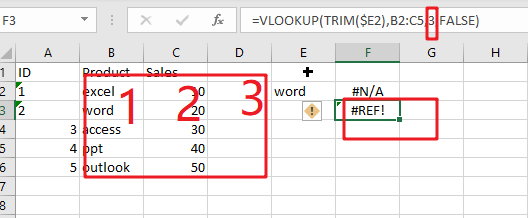### 3. VLOOKUP #VALUE Error

If you enter wrong data type in the VLOOKUP formula in Excel, and the #VALUE error will generate. And the below reasons will also cause this error.

• Index Number is less than 1

If your Index_number argument is less than 1 in VLOOKUP function, and it will return a #VALUE error.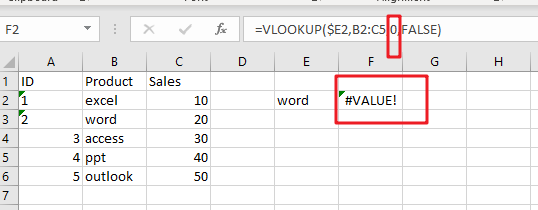• Lookup Value Length

You need to know that lookup value length should not be exceeded 256 characters, if lookup value character length exceeds this limit in VLOOKUP formula, and it returns a #VALUE error.

To fix this error, you can use INDEX function in combination with MATCH function to build another newly formula to achieve the same request.

### 4. Removing VLOOKUP Error Messages

If you want to make VLOOKUP errors clearer and easier to understand, and you can use the IFERROR function to display a meaningful message if VLOOKUP is not working and returns error.

`=IFERROR(VLOOKUP(\$E2,Sheet8!B2:C5,2,FALSE),"lookup value not found!!!")`

The IFERROR function can be used to check for any errors in the VLOOKUP formula, and if one VLOOKUP error message is returned by the VLOOKUP formula, excel will display “lookup value not found!!!” instead of any of the error messages, such as: #N/A,#REF,and #VALUE.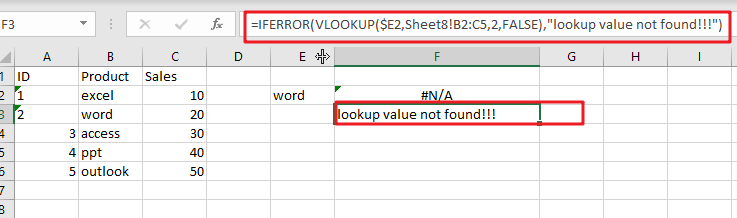Enjoy!

### Related Functions

• Excel IFERROR function
The Excel IFERROR function returns an alternate value you specify if a formula results in an error, or returns the result of the formula.The syntax of the IFERROR function is as below:= IFERROR (value, value_if_error)….
• Excel VLOOKUP function
The Excel VLOOKUP function lookup a value in the first column of the table and return the value in the same row based on index_num position.The syntax of the VLOOKUP function is as below:= VLOOKUP (lookup_value, table_array, column_index_num,[range_lookup])….
• Excel INDEX function
The Excel INDEX function returns a value from a table based on the index (row number and column number)The INDEX function is a build-in function in Microsoft Excel and it is categorized as a Lookup and Reference Function.The syntax of the INDEX function is as below:= INDEX (array, row_num,[column_num])…
• Excel MATCH  function
The Excel MATCH function search a value in an array and returns the position of that item.The MATCH function is a build-in function in Microsoft Excel and it is categorized as a Lookup and Reference Function.The syntax of the MATCH function is as below:= MATCH  (lookup_value, lookup_array, [match_type])….

## Fix #N/A Error For VLOOKUP From Another Sheet

This post will show you how to fix the #N/A error why it occurs when you extract values from another sheet using VLOOKUP function in Excel 2016,2013,2010 or other Excel versions. How can you correct a #N/A error in VLOOKUP function in Excel. This post will also show you several reasons why VLOOKUP formula is not working and displaying the #N/A, #NAME and #VALUE error messages.

## The Reasons Why VLOOKUP Formula is Not Working

#1 Lookup value is not in the first column of the table array

The VLOOKUP function is that it can only look for values on the left-most column in the table array and if lookup value is not in the first column of the array, and you will see the #N/A error.

Assume that you want to retrieve the number of sales for product “word”, and you can use the following VLOOKUP function:

`=VLOOKUP(E2,A2:C6,2,FALSE)`

You should see that the #N/A error message will be shown, because the lookup value “word” in Cell E2 appears in the second column of the table array argument A2:C6.

To correct the #N/A error, and you need to adjust your VLOOKUP to reference the correct column.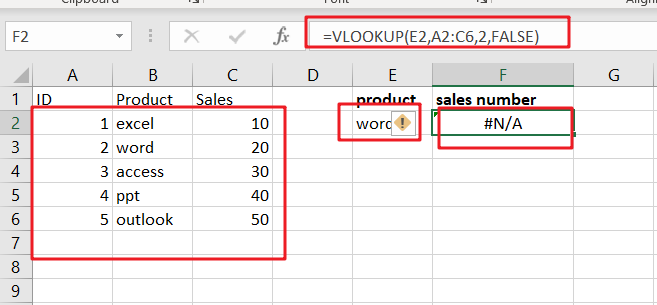#2 #N/A Error in Exact Match VLOOKUP

If you are starting with an exact match and the exact value “Words” is not found, and the #N/A error is returned. Type the following VLOOKUP formula in Cell F2:

`=VLOOKUP("Words",B2:C5,2,FALSE)`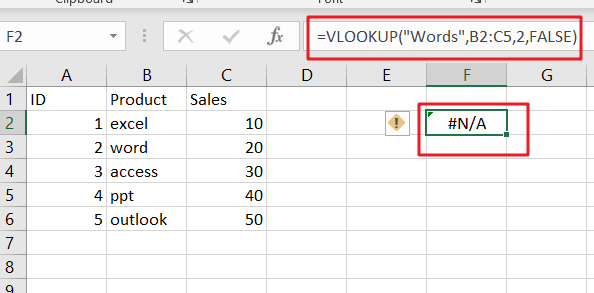#3 #N/A Error in Approximate Match VLOOKUP

If you are starting with an approximate match using VLOOKUP function, and your formula may be return the #N/A error message caused by the following two cases:

If the lookup column is not sorted in ascending order.

If the lookup value is smaller than the smallest value in the lookup array.

## Remove VLOOKUP #N/A Error

When using VLOOKUP function and it can not find any data or when you haven’t entered a lookup value in VLOOKUP formula, and you should get an #N/A error message. And this error message is not helpful at all. You may be want to remove it in your worksheet. And you can use the IFERROR function to remove #N/A error message in VLOOKUP function.

You can put the above VLOOKUP function into the IFERROR function as a new formula, refer to the below formula based on the IFERROR function and VLOOKUP function, it returns a blank string instead of #N/A error.

`=IFERROR(VLOOKUP("Words",B2:C5,2,FALSE),"")`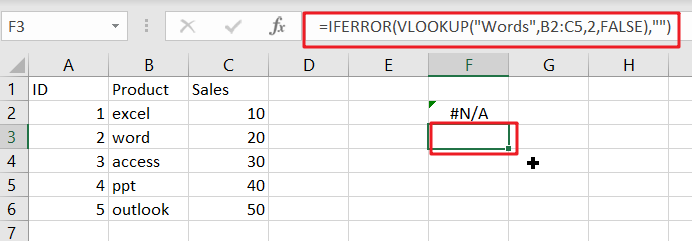If you are using Excel 2003, and you can use the IF function and the ISERROR function, as the IFERROR function is not available in Excel 2003, type:

`=IF(ISERROR(VLOOKUP("Words",B2:C5,2,FALSE)),"",VLOOKUP("Words",B2:C5,2,FALSE))`

You need to put the VLOOKUP function in there twice to remove #N/A error message in Excel 2003.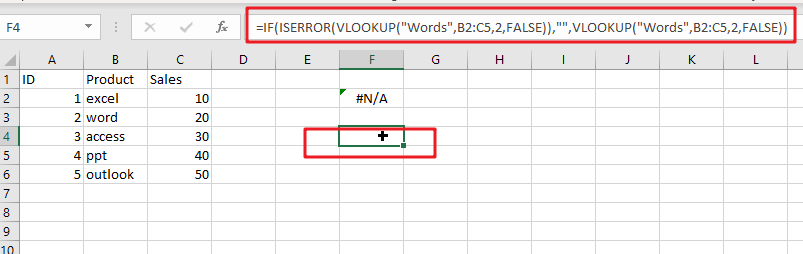### Related Functions

• Excel IF function
The Excel IF function perform a logical test to return one value if the condition is TRUE and return another value if the condition is FALSE. The IF function is a build-in function in Microsoft Excel and it is categorized as a Logical Function.The syntax of the IF function is as below:= IF (condition, [true_value], [false_value])….
• Excel ISERROR function
The Excel ISERROR function used to check for any error type that excel generates and it returns TRUE for any error type, and the ISERR function also can be checked for error values except #N/A error, it returns TRUE while the error is #N/A. The syntax of the ISERROR function is as below:= ISERROR (value)….
• Excel IFERROR function
The Excel IFERROR function returns an alternate value you specify if a formula results in an error, or returns the result of the formula.The syntax of the IFERROR function is as below:= IFERROR (value, value_if_error)….
• Excel VLOOKUP function
The Excel VLOOKUP function lookup a value in the first column of the table and return the value in the same row based on index_num position.The syntax of the VLOOKUP function is as below:= VLOOKUP (lookup_value, table_array, column_index_num,[range_lookup])….